# Photometric stereo http www cs cmu edu16385 16

• Slides: 90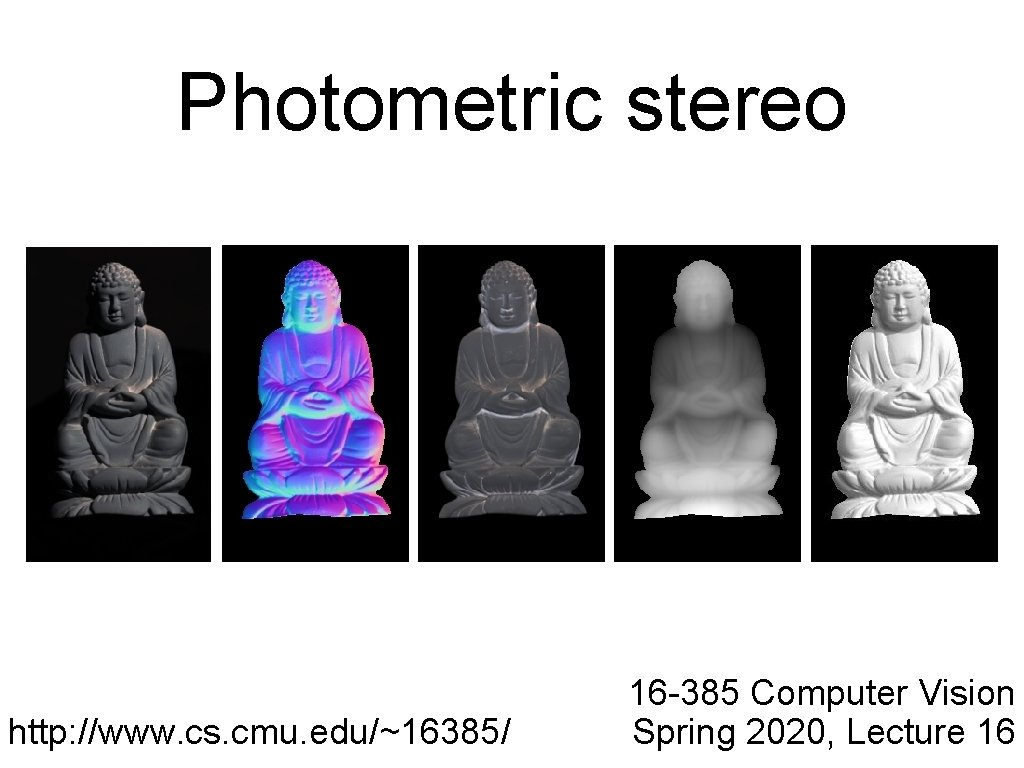Photometric stereo http: //www. cs. cmu. edu/~16385/ 16 -385 Computer Vision Spring 2020, Lecture 16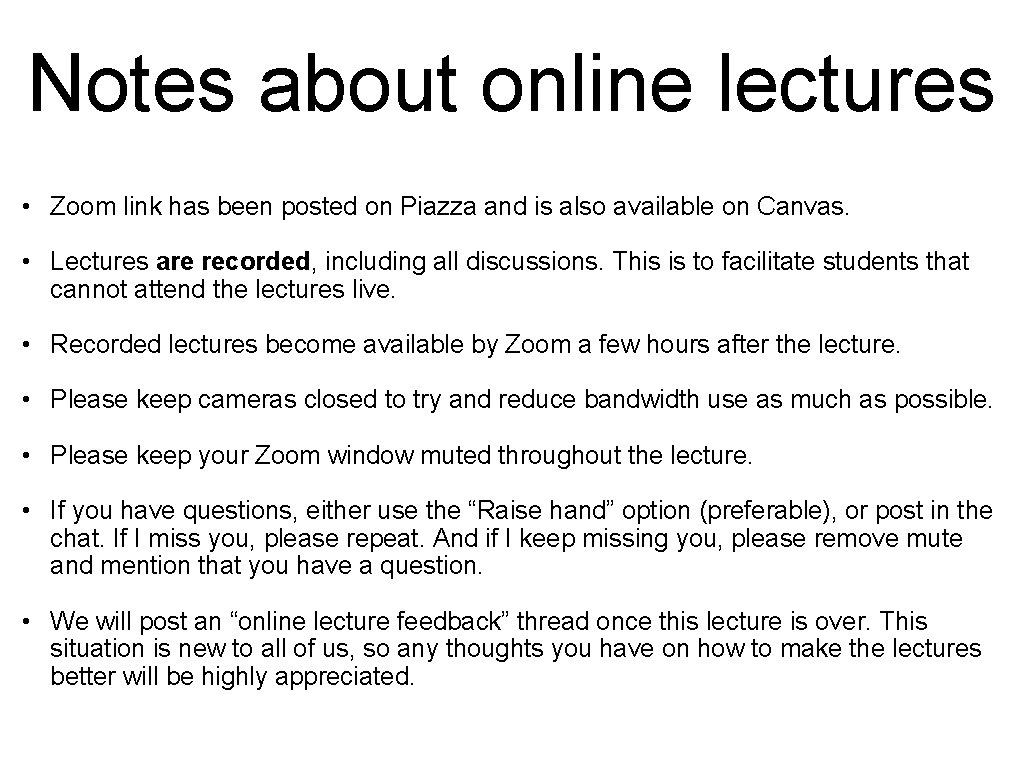Notes about online lectures • Zoom link has been posted on Piazza and is also available on Canvas. • Lectures are recorded, including all discussions. This is to facilitate students that cannot attend the lectures live. • Recorded lectures become available by Zoom a few hours after the lecture. • Please keep cameras closed to try and reduce bandwidth use as much as possible. • Please keep your Zoom window muted throughout the lecture. • If you have questions, either use the “Raise hand” option (preferable), or post in the chat. If I miss you, please repeat. And if I keep missing you, please remove mute and mention that you have a question. • We will post an “online lecture feedback” thread once this lecture is over. This situation is new to all of us, so any thoughts you have on how to make the lectures better will be highly appreciated.Notes about office hours • Regular office hours on Mondays, Tuesdays, and Fridays continue. • Throughout the semester, there additional office hours on Thursdays, 4 -6 pm. • We will post updated Zoom links for office hours on Piazza.Notes about homework logistics • Take-home quizzes have been reduced to 11. You can still skip 3 of them. • Take-home quiz 6 is due on Sunday, March 22. • Programming assignment 4 was posted yesterday and is due on Wednesday, March 25. • Programming assignment schedule has been shifted, as shown on the course website. • Last programming assignment will need to be a little shorter, to fit within 1. 5 weeks instead of the usual two.Questions?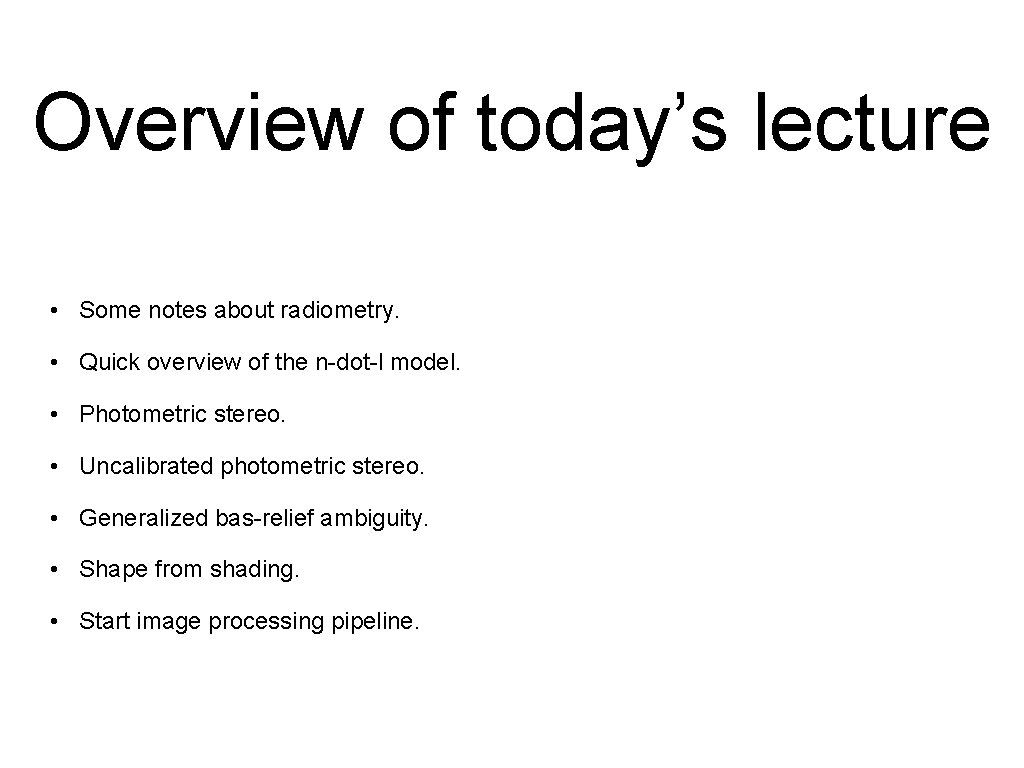Overview of today’s lecture • Some notes about radiometry. • Quick overview of the n-dot-l model. • Photometric stereo. • Uncalibrated photometric stereo. • Generalized bas-relief ambiguity. • Shape from shading. • Start image processing pipeline.Slide credits Many of these slides were adapted from: • Srinivasa Narasimhan (16 -385, Spring 2014). • Todd Zickler (Harvard University). • Steven Gortler (Harvard University). • Kayvon Fatahalian (Stanford University; CMU 15 -462, Fall 2015).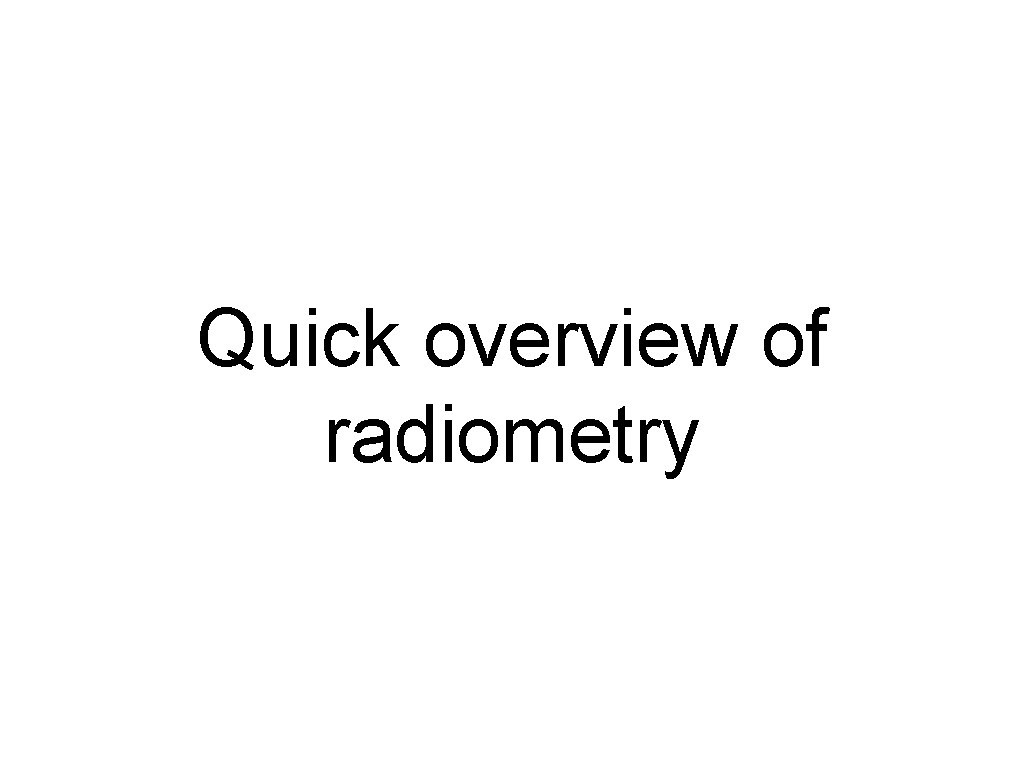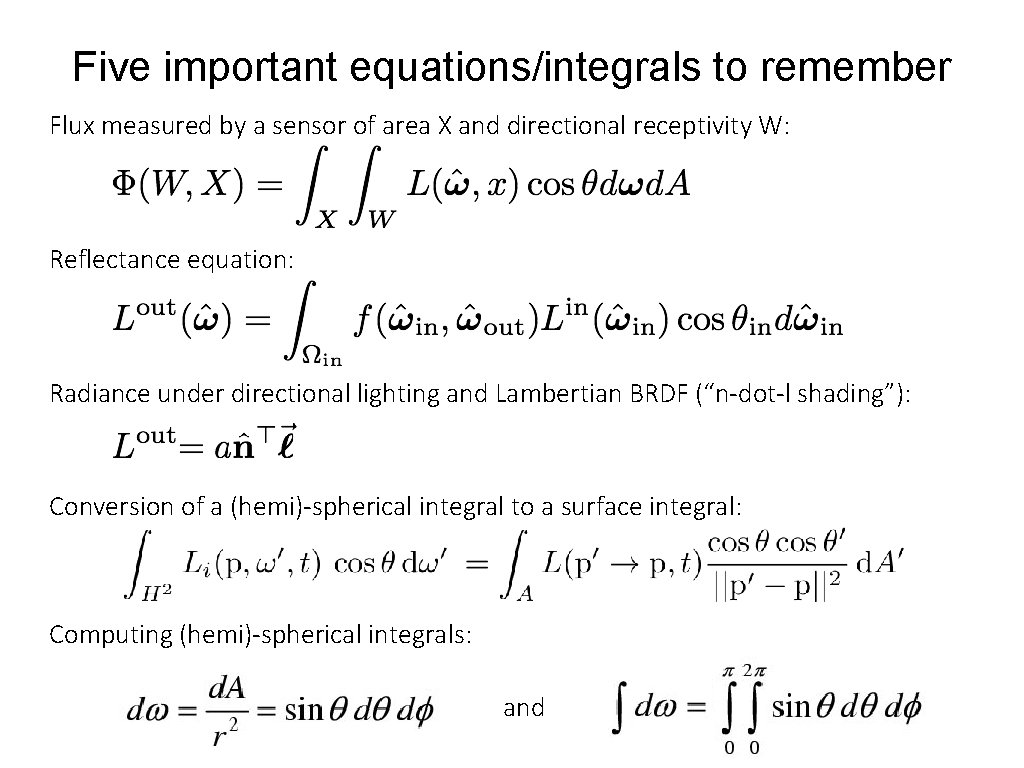Five important equations/integrals to remember Flux measured by a sensor of area X and directional receptivity W: Reflectance equation: Radiance under directional lighting and Lambertian BRDF (“n-dot-l shading”): Conversion of a (hemi)-spherical integral to a surface integral: Computing (hemi)-spherical integrals: and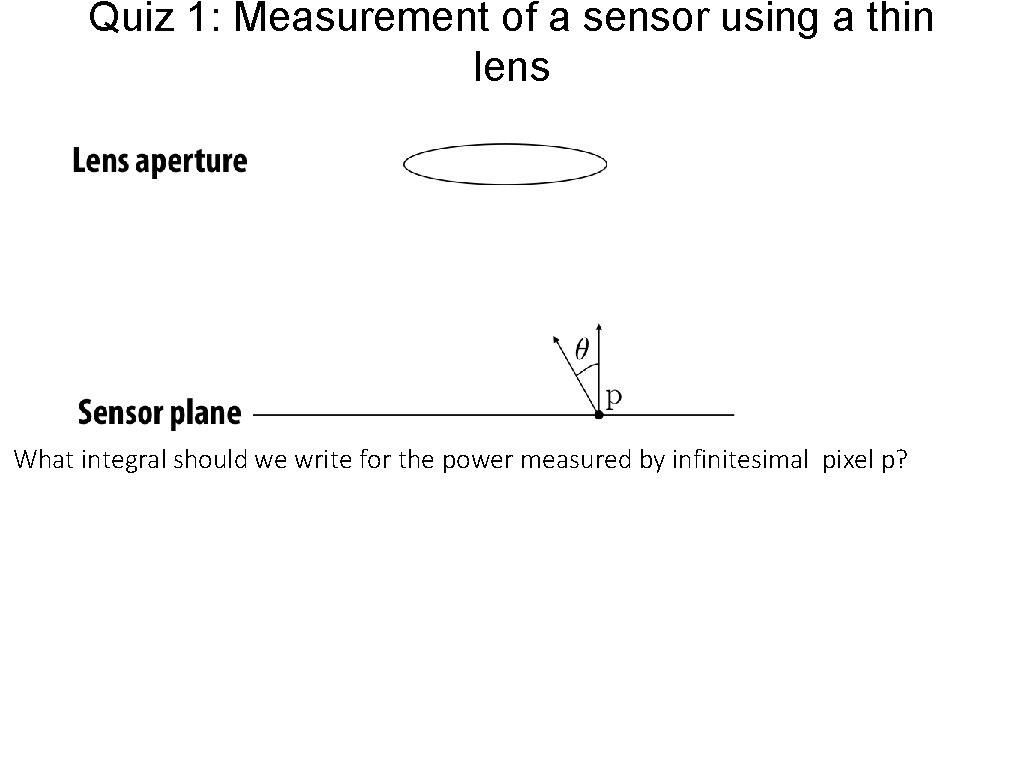Quiz 1: Measurement of a sensor using a thin lens What integral should we write for the power measured by infinitesimal pixel p?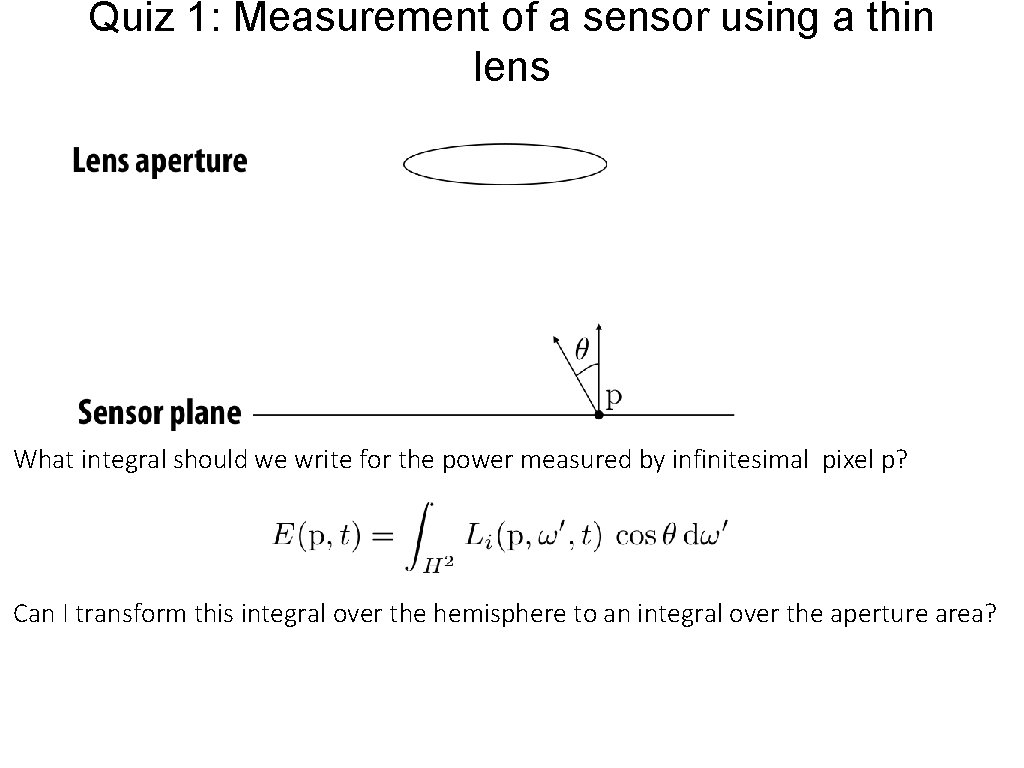Quiz 1: Measurement of a sensor using a thin lens What integral should we write for the power measured by infinitesimal pixel p? Can I transform this integral over the hemisphere to an integral over the aperture area?Quiz 1: Measurement of a sensor using a thin lens What integral should we write for the power measured by infinitesimal pixel p? Can I transform this integral over the hemisphere to an integral over the aperture area?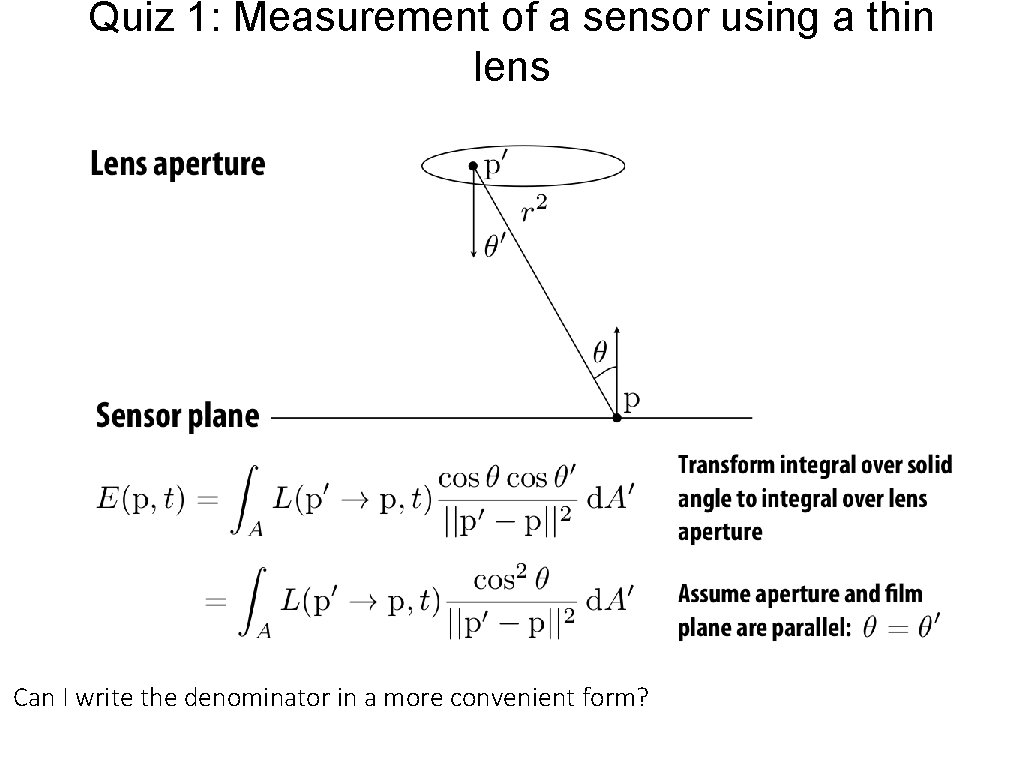Quiz 1: Measurement of a sensor using a thin lens Can I write the denominator in a more convenient form?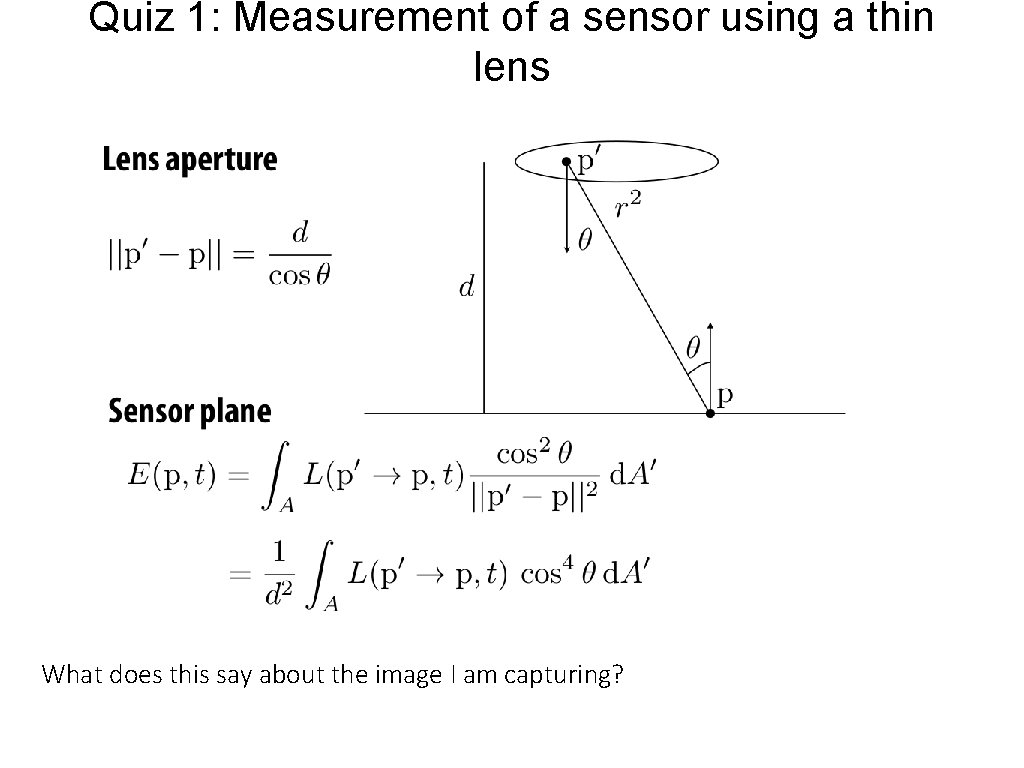Quiz 1: Measurement of a sensor using a thin lens What does this say about the image I am capturing?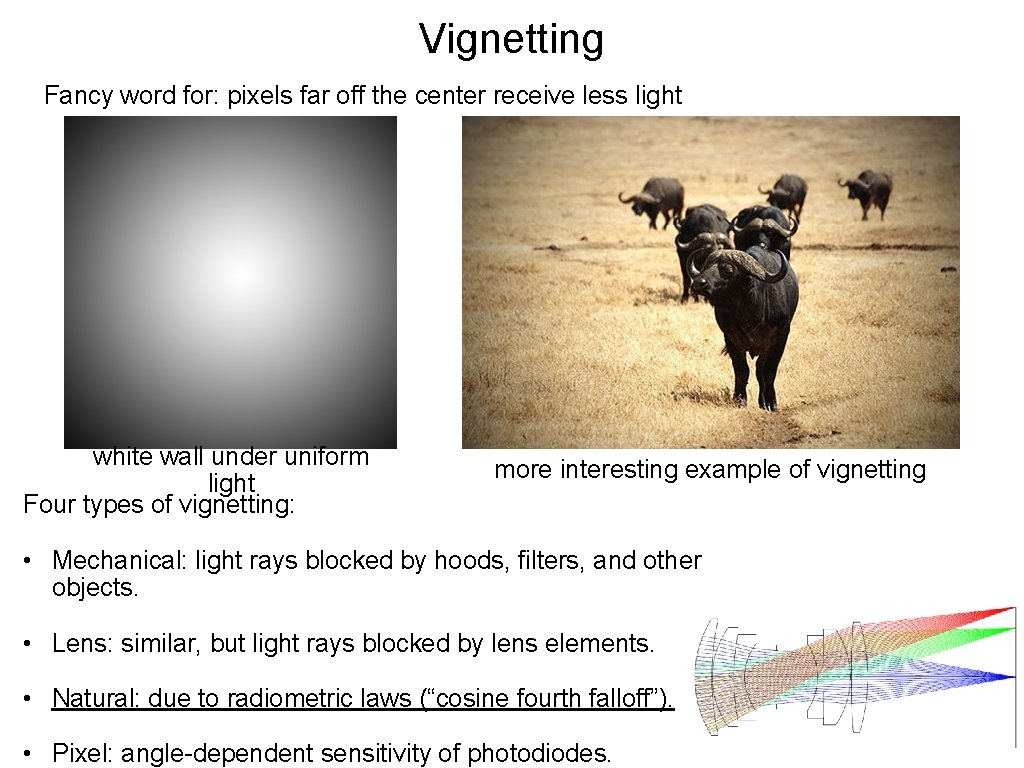Vignetting Fancy word for: pixels far off the center receive less light white wall under uniform light Four types of vignetting: more interesting example of vignetting • Mechanical: light rays blocked by hoods, filters, and other objects. • Lens: similar, but light rays blocked by lens elements. • Natural: due to radiometric laws (“cosine fourth falloff”). • Pixel: angle-dependent sensitivity of photodiodes.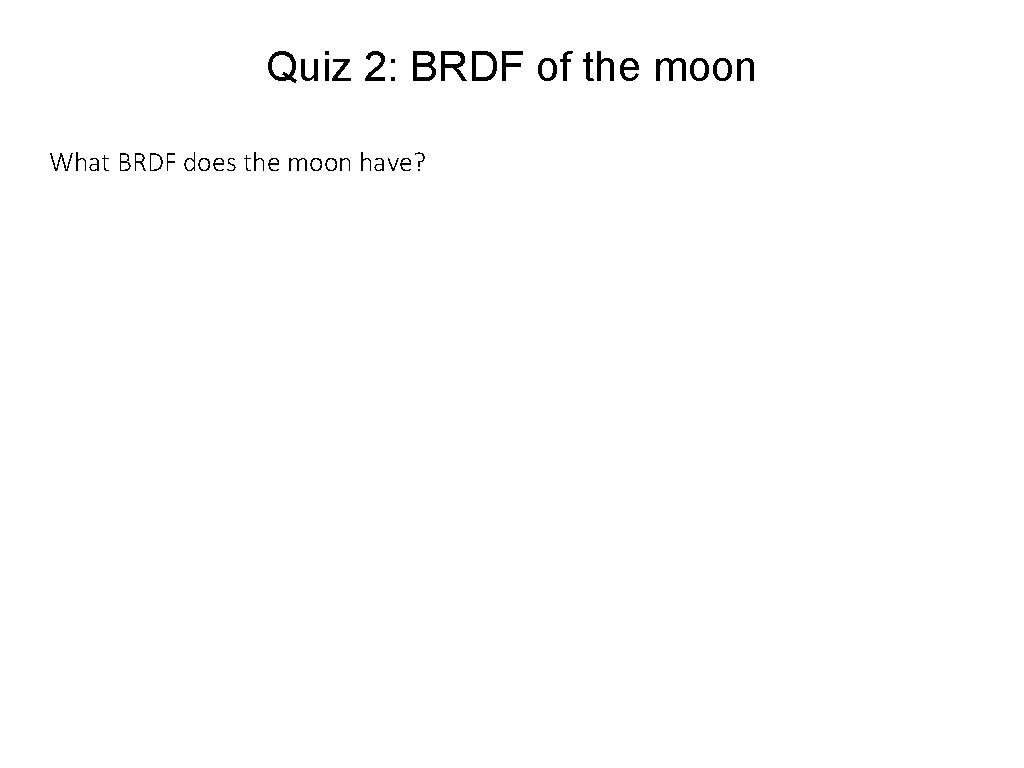Quiz 2: BRDF of the moon What BRDF does the moon have?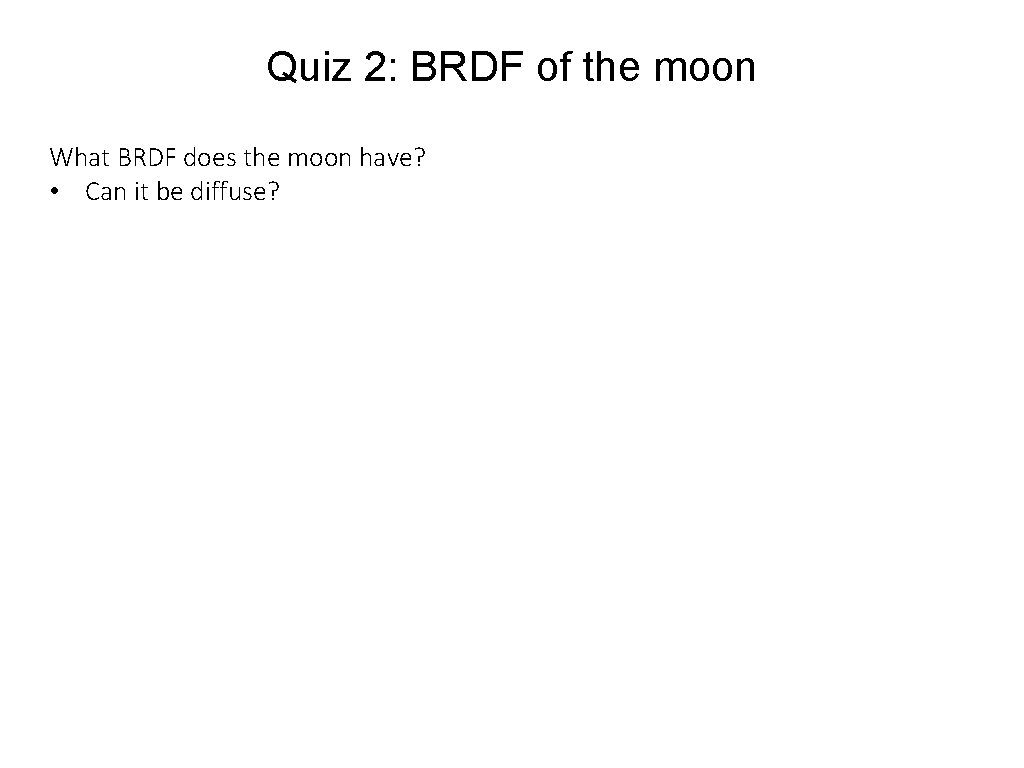Quiz 2: BRDF of the moon What BRDF does the moon have? • Can it be diffuse?Quiz 2: BRDF of the moon What BRDF does the moon have? • Can it be diffuse? Even though the moon appears matte, its edges remain bright.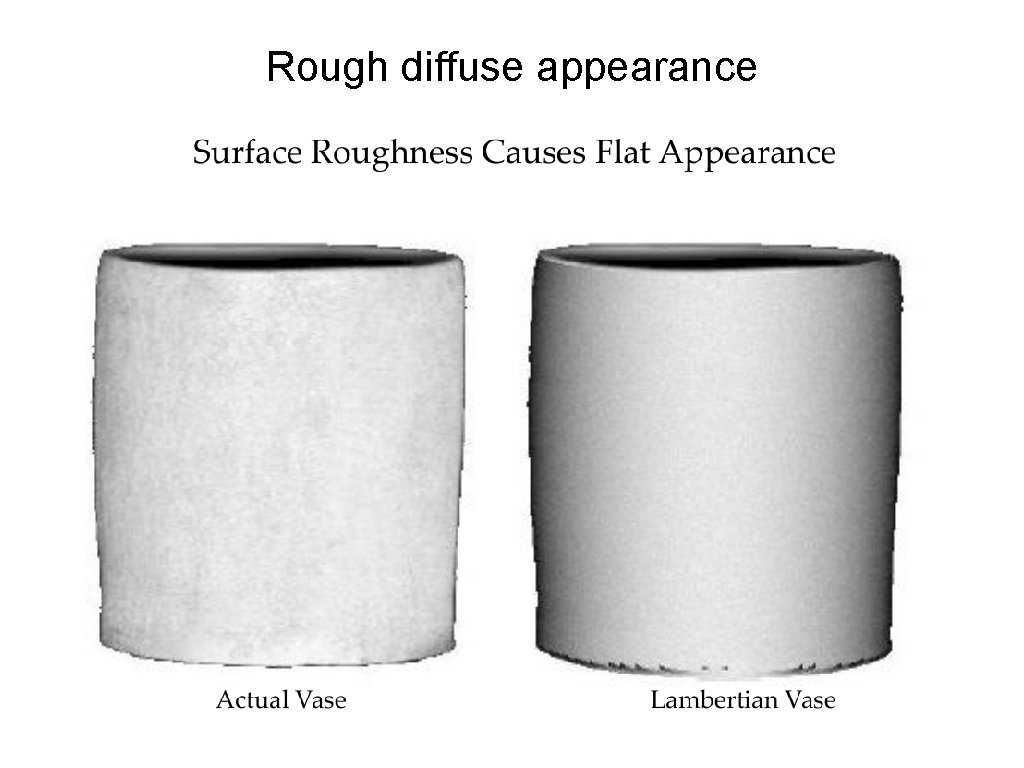Rough diffuse appearance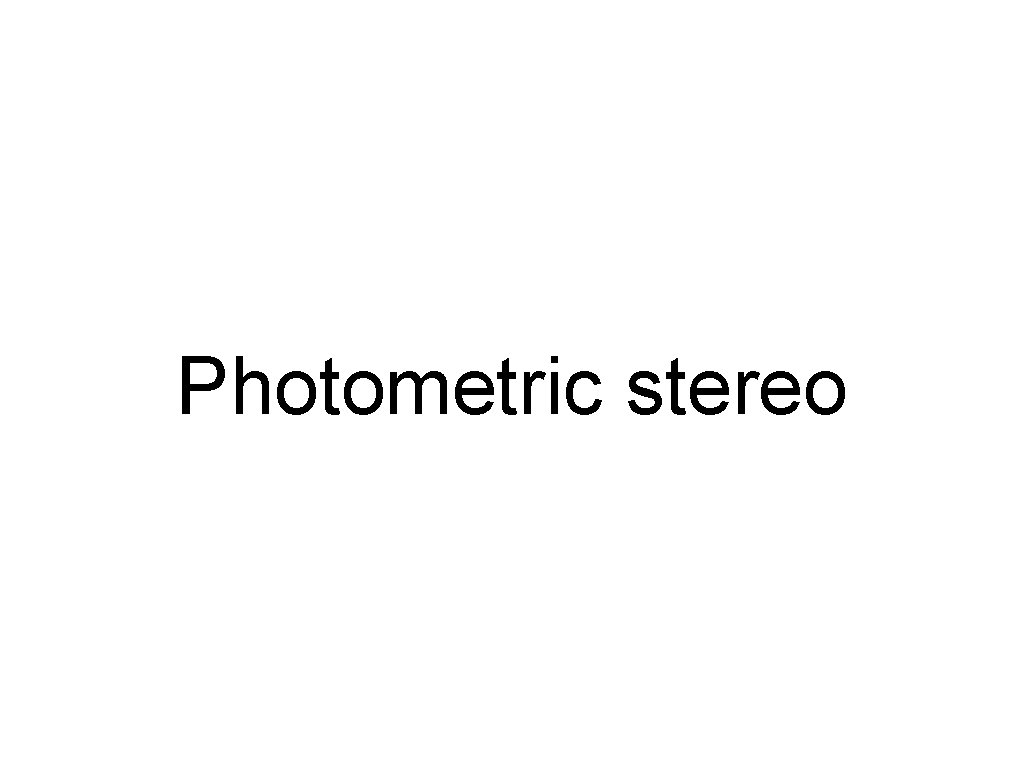Photometric stereo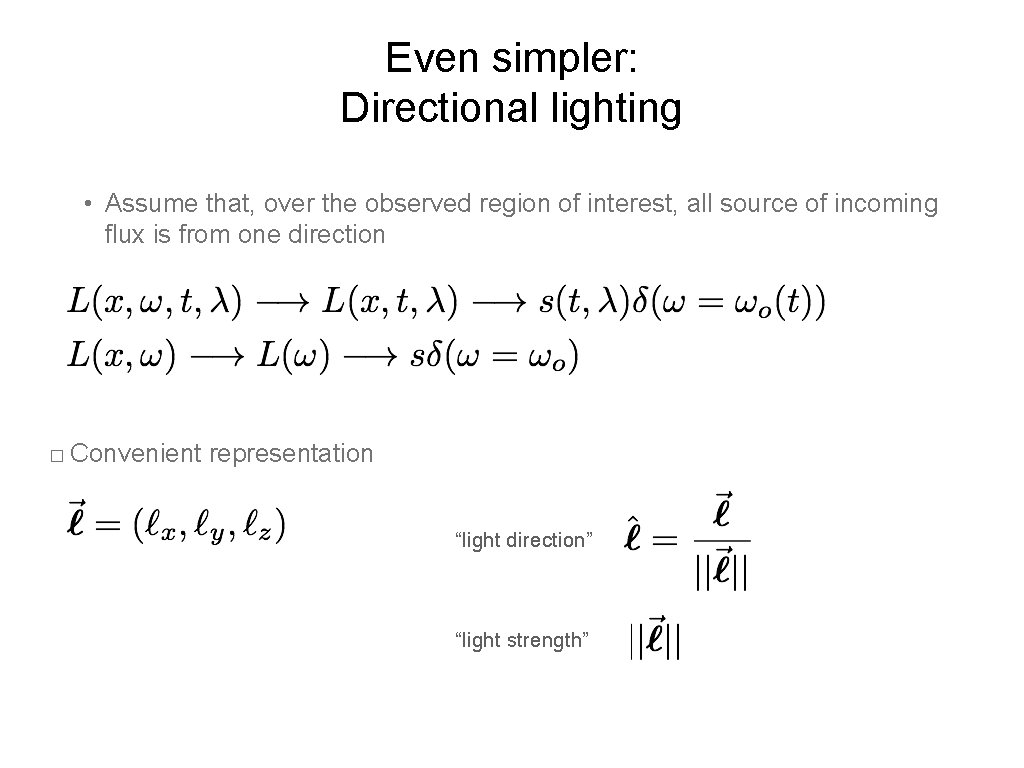Even simpler: Directional lighting • Assume that, over the observed region of interest, all source of incoming flux is from one direction � Convenient representation “light direction” “light strength”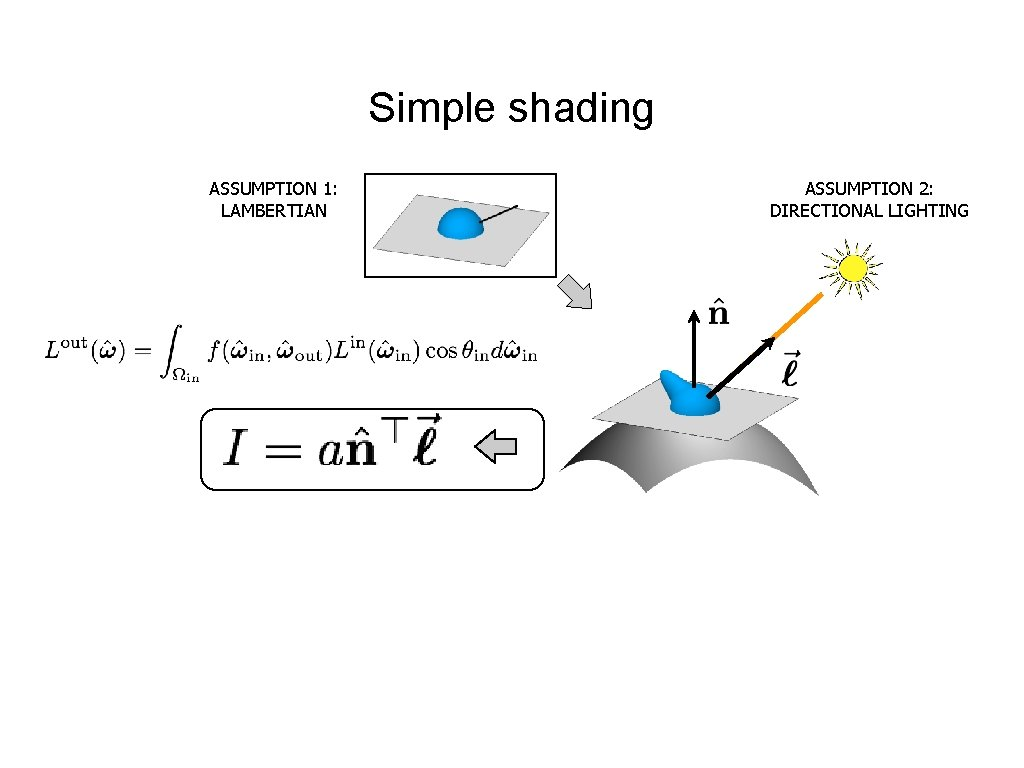Simple shading ASSUMPTION 1: LAMBERTIAN ASSUMPTION 2: DIRECTIONAL LIGHTING“N-dot-l” shading ASSUMPTION 1: LAMBERTIAN ASSUMPTION 2: DIRECTIONAL LIGHTINGImage Intensity and 3 D Geometry • Shading as a cue for shape reconstruction • What is the relation between intensity and shape?“N-dot-l” shading ASSUMPTION 1: LAMBERTIAN ASSUMPTION 2: DIRECTIONAL LIGHTING Why do we call these normal “shape”?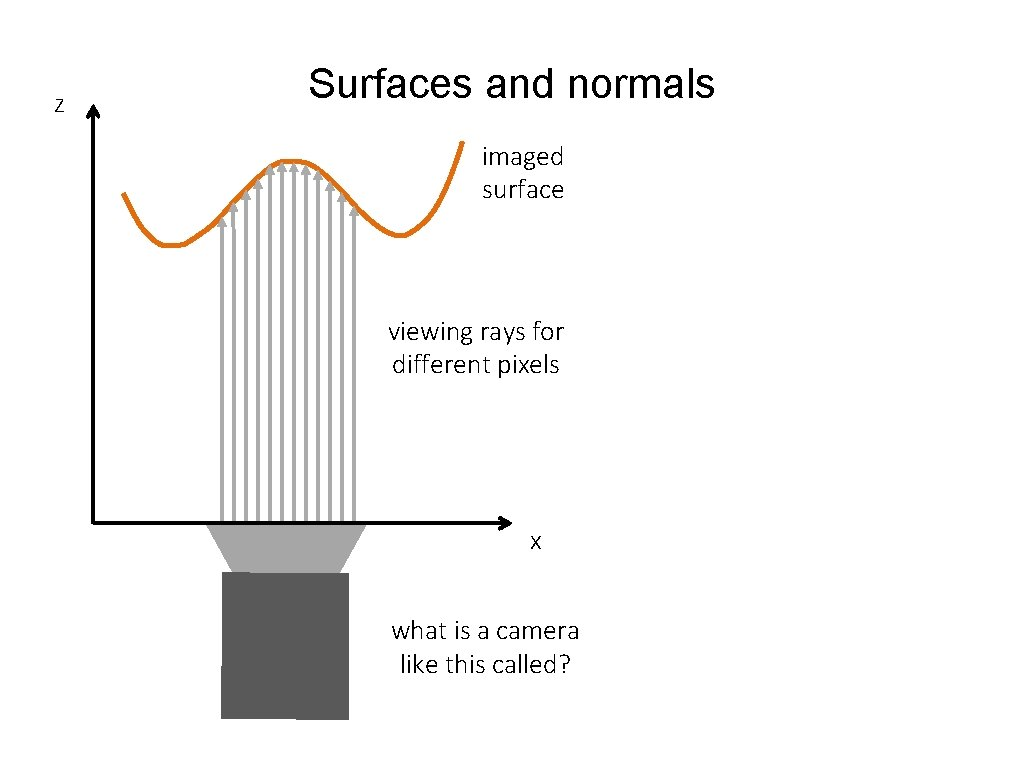z Surfaces and normals imaged surface viewing rays for different pixels x what is a camera like this called?z Surfaces and normals imaged surface Surface representation as a depth field (also known as Monge surface): viewing rays for different pixels pixel coordinates on image place depth at each pixel x orthographic camera How does surface normal relate to this representation?z Surfaces and normals imaged surface Surface representation as a depth image (also known as Monge surface): viewing rays for different pixels pixel coordinates on image place depth at each pixel Unnormalized normal: x orthographic camera Actual normal: Normals are scaled spatial derivatives of depth image!Shape from a Single Image? • Given a single image of an object with known surface reflectance taken under a known light source, can we recover the shape of the object?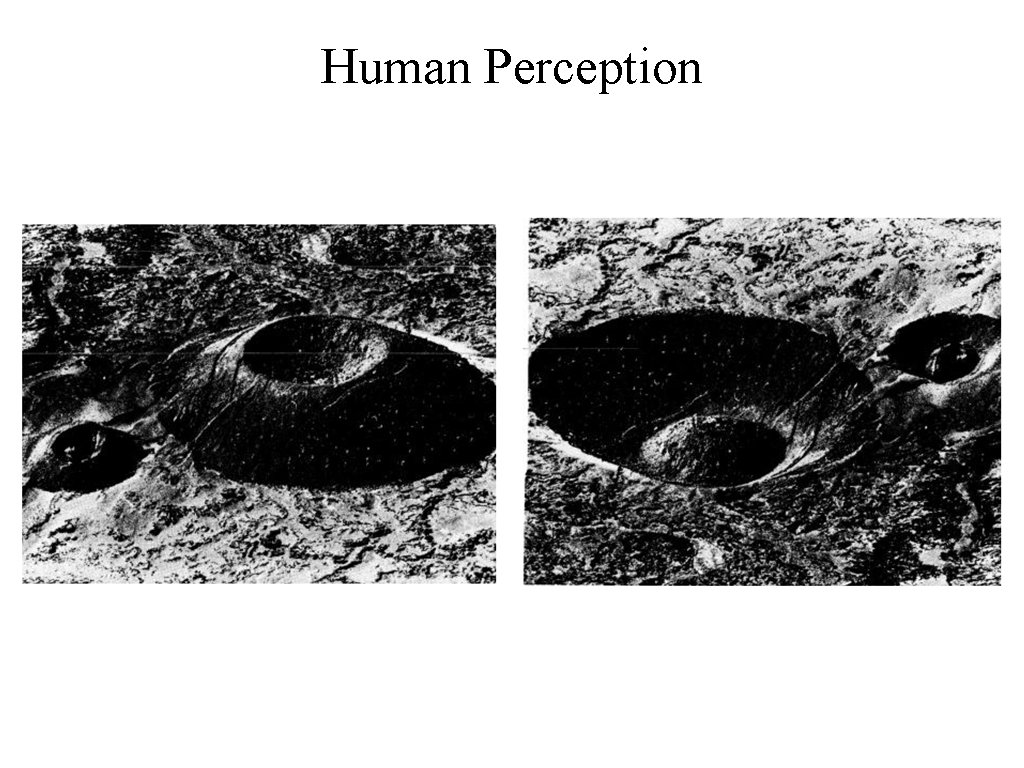Human PerceptionExamples of the classic bump/dent stimuli used to test lighting assumptions when judging shape from shading, with shading orientations (a) 0° and (b) 180° from the vertical. Thomas R et al. J Vis 2010; 10: 6Human Perception • Our brain often perceives shape from shading. • Mostly, it makes many assumptions to do so. • For example: Light is coming from above (sun). Biased by occluding contours. by V. RamachandranSingle-lighting is ambiguous ASSUMPTION 1: LAMBERTIAN ASSUMPTION 2: DIRECTIONAL LIGHTING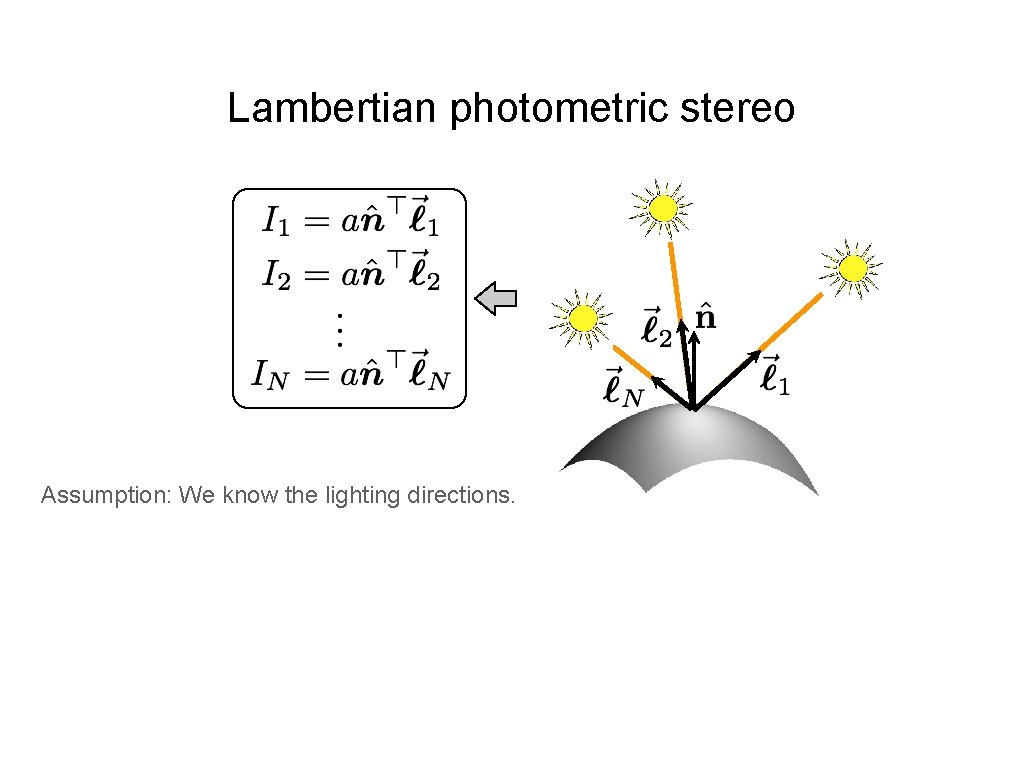Lambertian photometric stereo Assumption: We know the lighting directions.Lambertian photometric stereo define “pseudo-normal” solve linear system for pseudo-normal What are the dimensions of these matrices?Lambertian photometric stereo define “pseudo-normal” solve linear system for pseudo-normal What are the knowns and unknowns?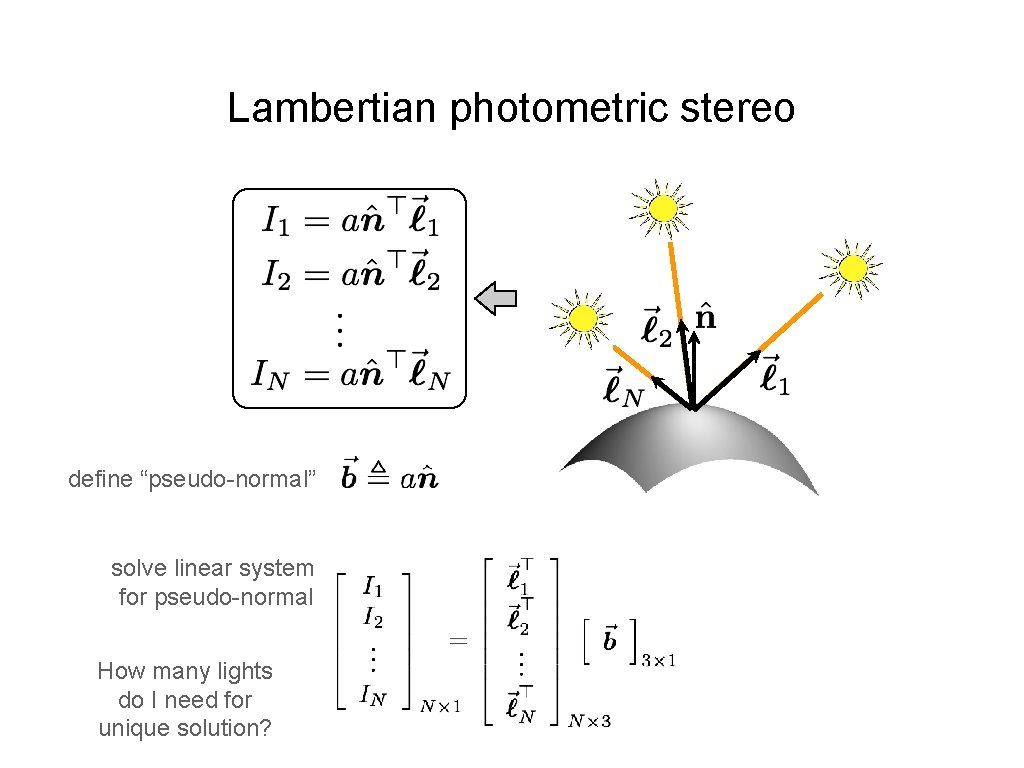Lambertian photometric stereo define “pseudo-normal” solve linear system for pseudo-normal How many lights do I need for unique solution?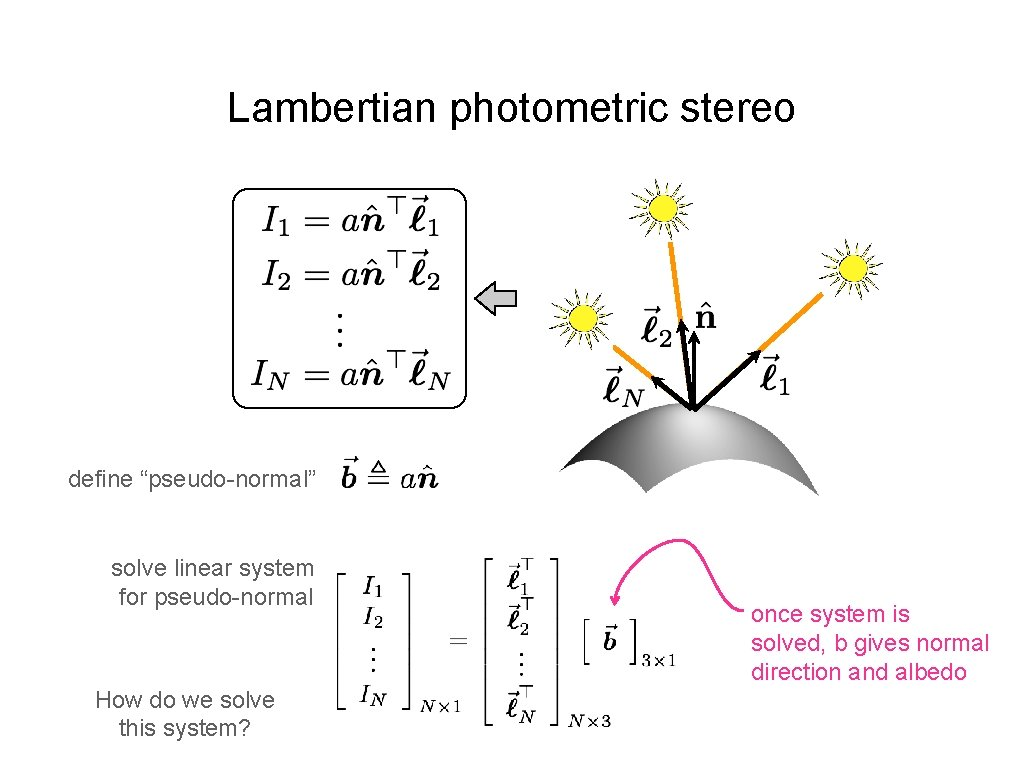Lambertian photometric stereo define “pseudo-normal” solve linear system for pseudo-normal How do we solve this system? once system is solved, b gives normal direction and albedoSolving the Equation with three lights inverse Is there any reason to use more than three lights?More than Three Light Sources • Get better SNR by using more lights • Least squares solution: • Solve for as before Moore-Penrose pseudo inverseComputing light source directions • Trick: place a chrome sphere in the scene – the location of the highlight tells you the source directionLimitations • Big problems – Doesn’t work for shiny things, semi-translucent things – Shadows, inter-reflections • Smaller problems – Camera and lights have to be distant – Calibration requirements • • measure light source directions, intensities camera response function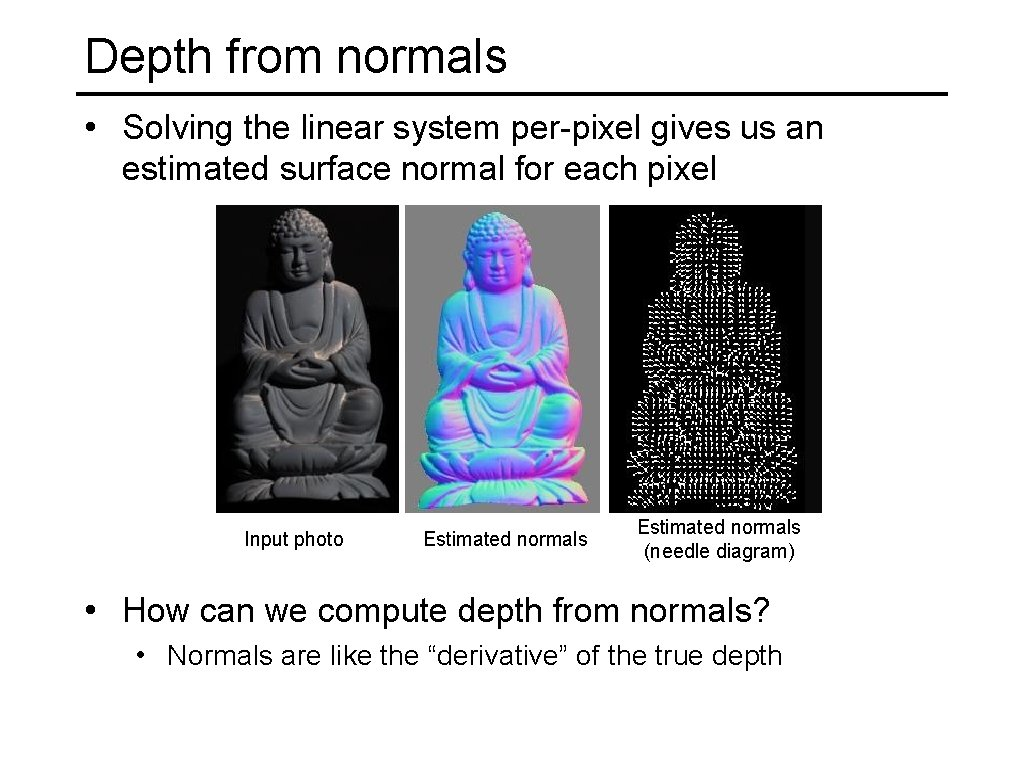Depth from normals • Solving the linear system per-pixel gives us an estimated surface normal for each pixel Input photo Estimated normals (needle diagram) • How can we compute depth from normals? • Normals are like the “derivative” of the true depth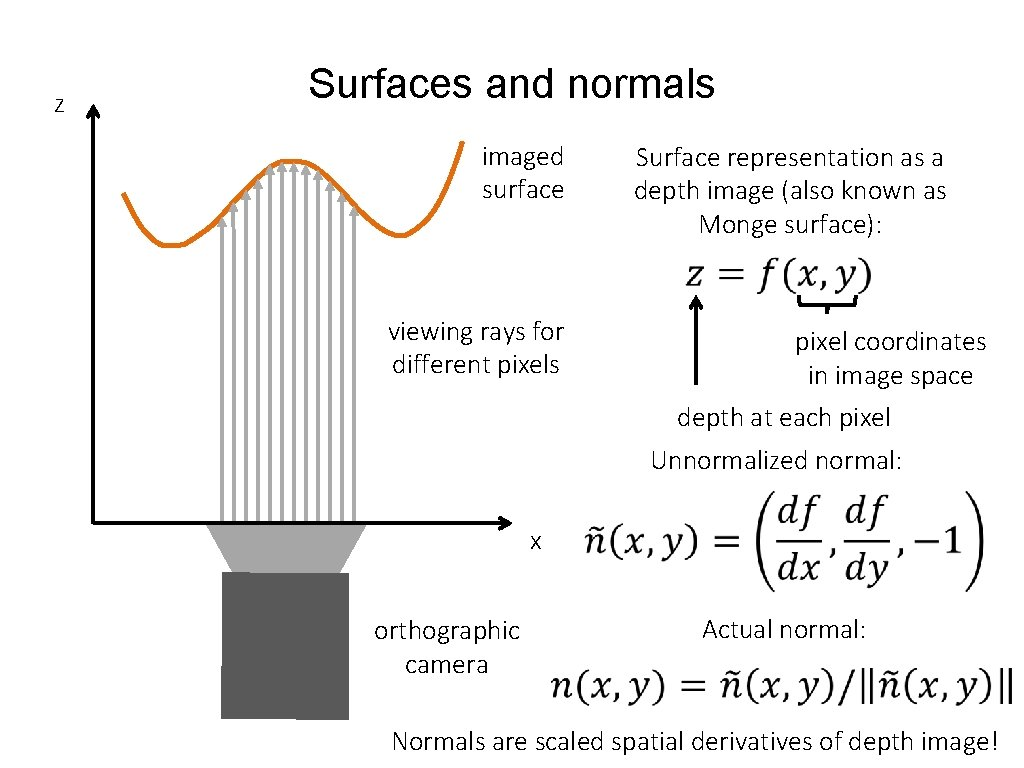z Surfaces and normals imaged surface Surface representation as a depth image (also known as Monge surface): viewing rays for different pixels pixel coordinates in image space depth at each pixel Unnormalized normal: x orthographic camera Actual normal: Normals are scaled spatial derivatives of depth image!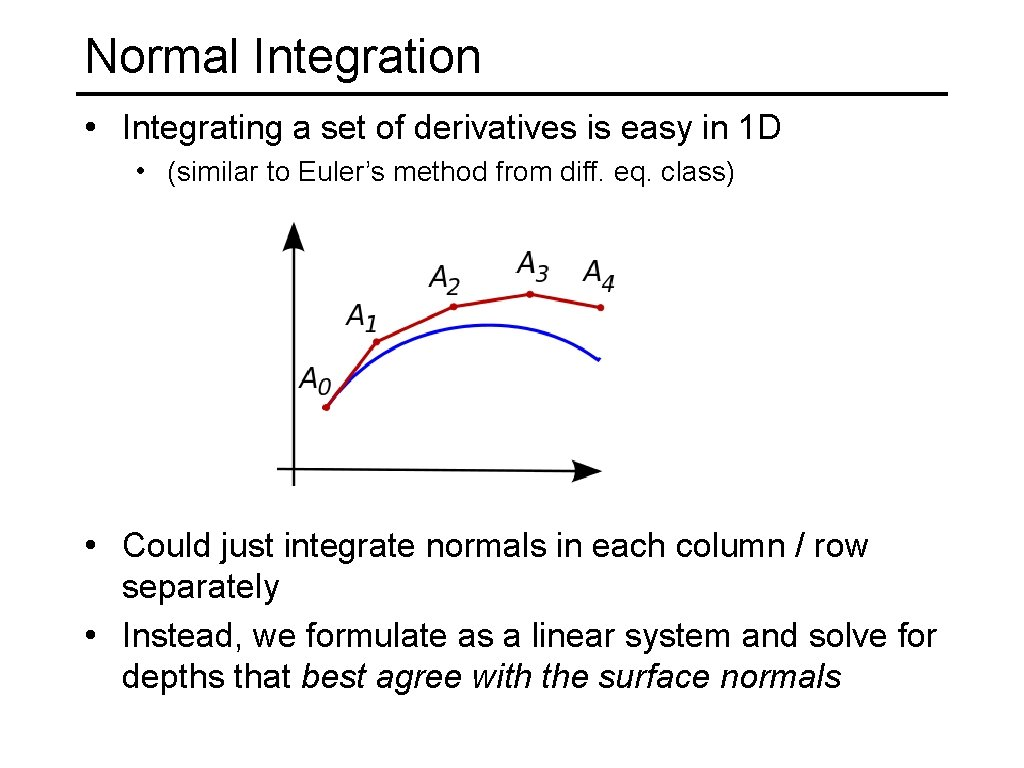Normal Integration • Integrating a set of derivatives is easy in 1 D • (similar to Euler’s method from diff. eq. class) • Could just integrate normals in each column / row separately • Instead, we formulate as a linear system and solve for depths that best agree with the surface normals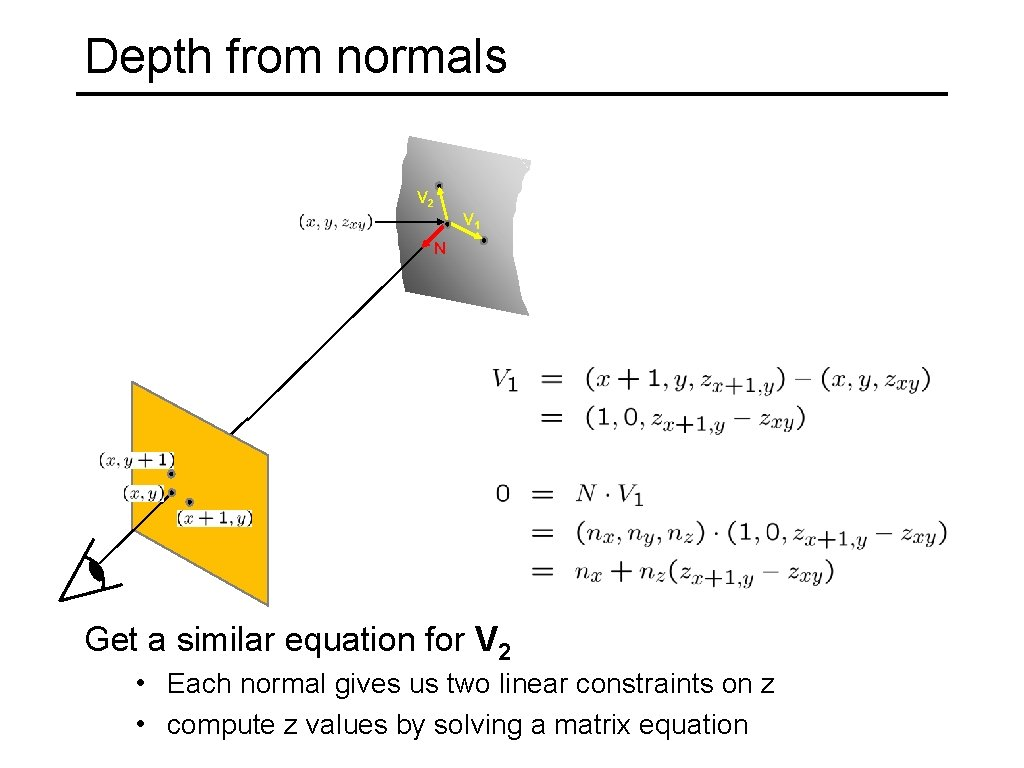Depth from normals V 2 V 1 N Get a similar equation for V 2 • Each normal gives us two linear constraints on z • compute z values by solving a matrix equation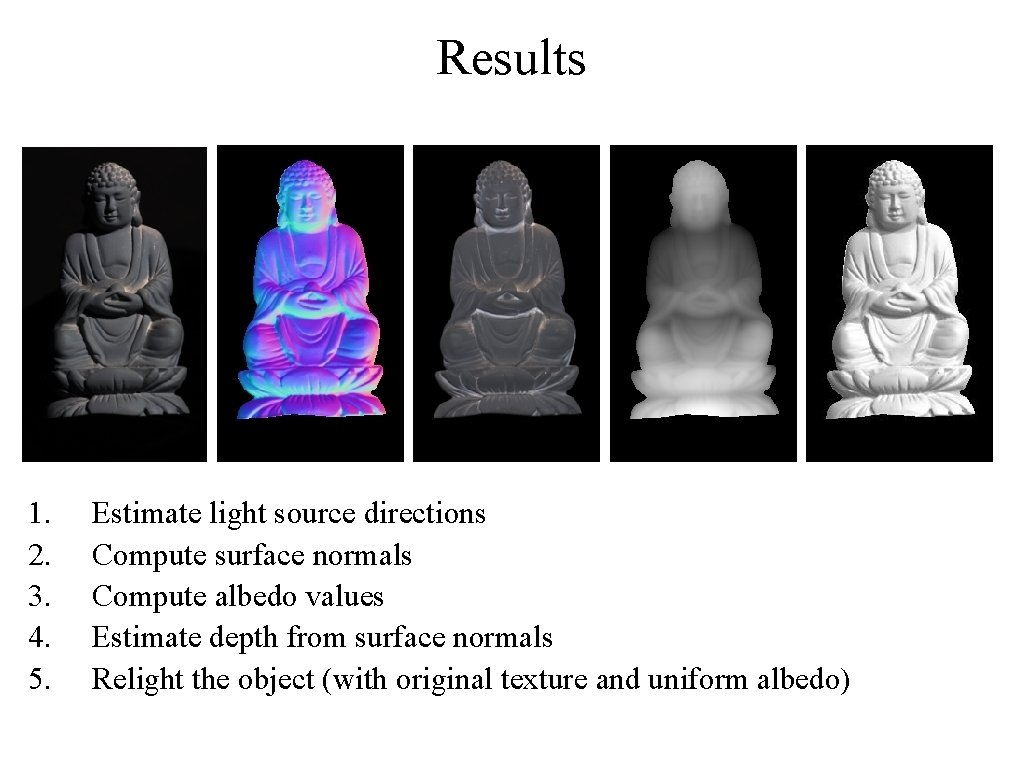Results 1. 2. 3. 4. 5. Estimate light source directions Compute surface normals Compute albedo values Estimate depth from surface normals Relight the object (with original texture and uniform albedo)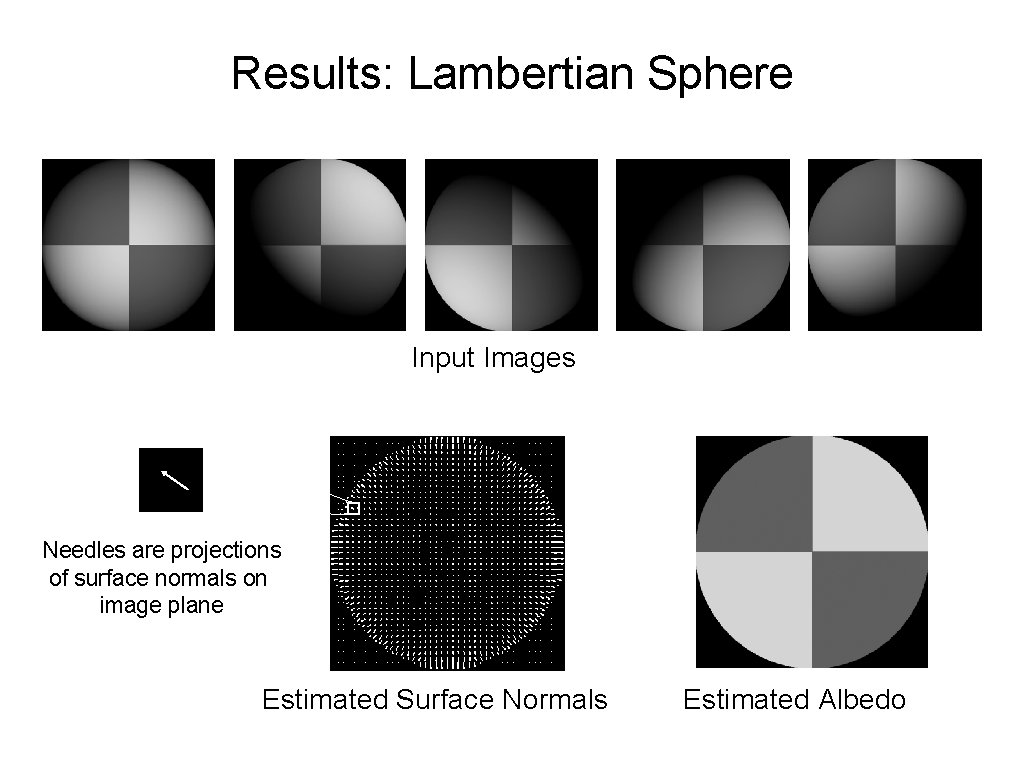Results: Lambertian Sphere Input Images Needles are projections of surface normals on image plane Estimated Surface Normals Estimated AlbedoResults – Albedo and Surface Normal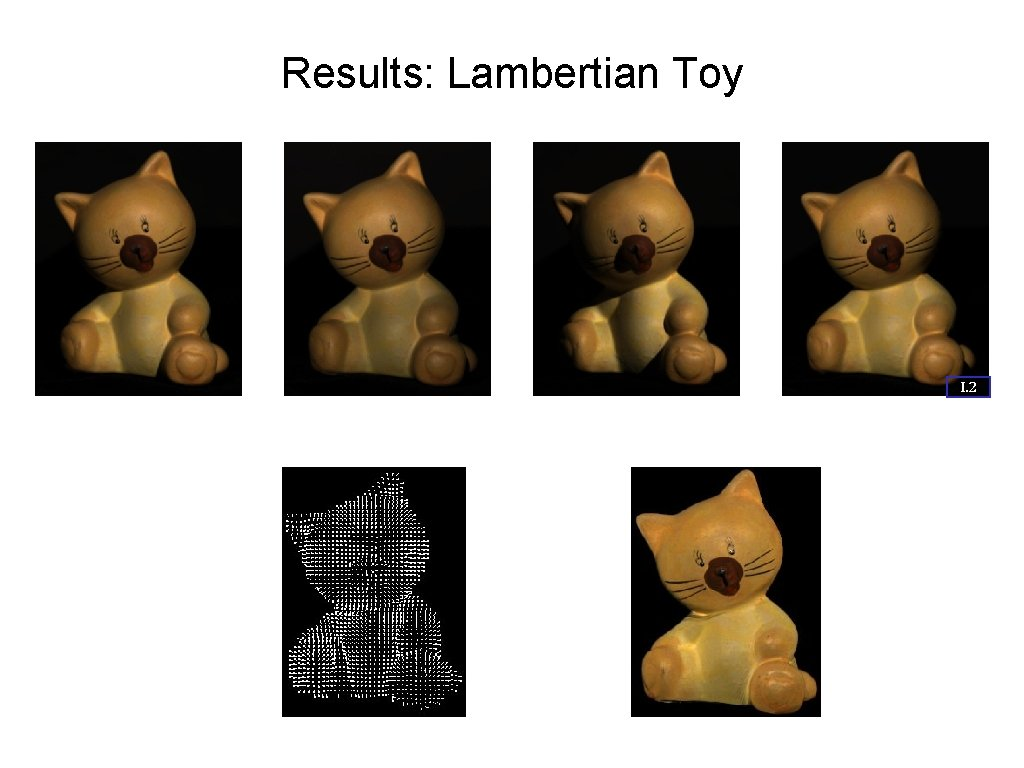Results: Lambertian Toy I. 2 Input Images Estimated Surface Normals Estimated Albedo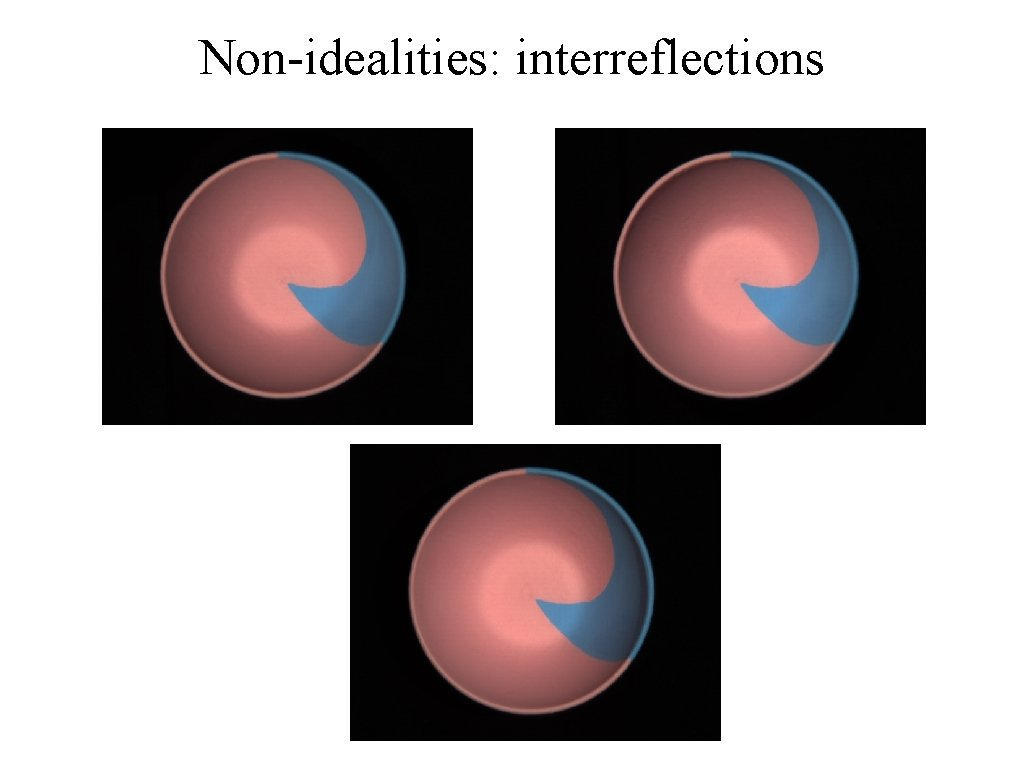Non-idealities: interreflections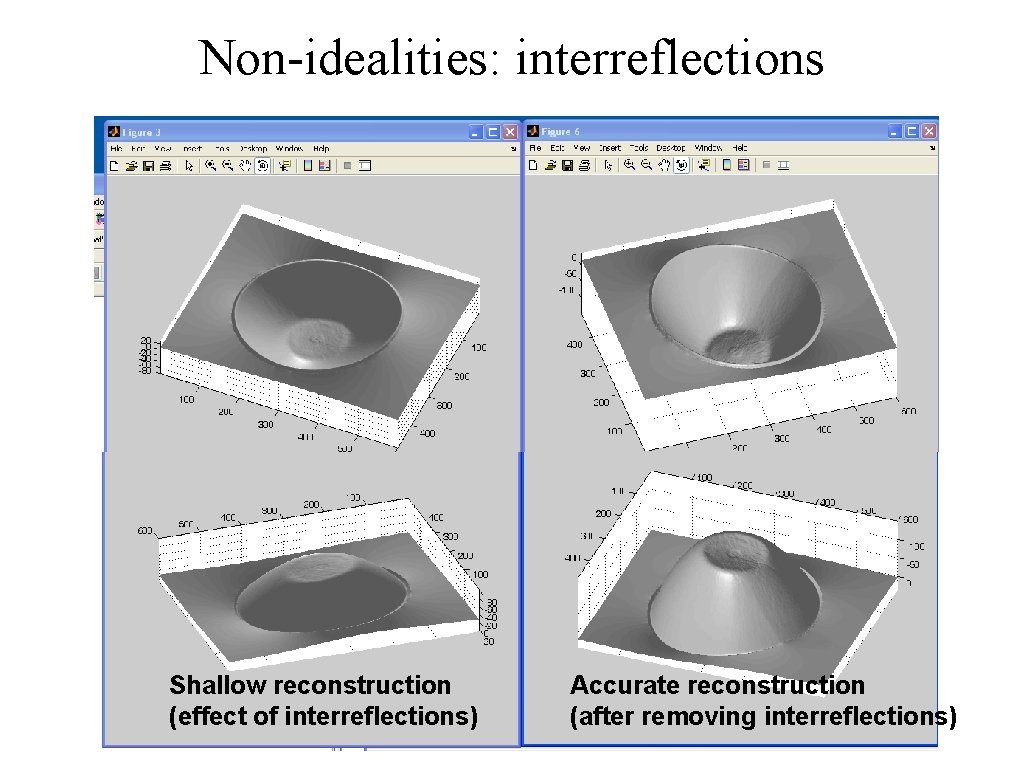Non-idealities: interreflections Shallow reconstruction (effect of interreflections) Accurate reconstruction (after removing interreflections)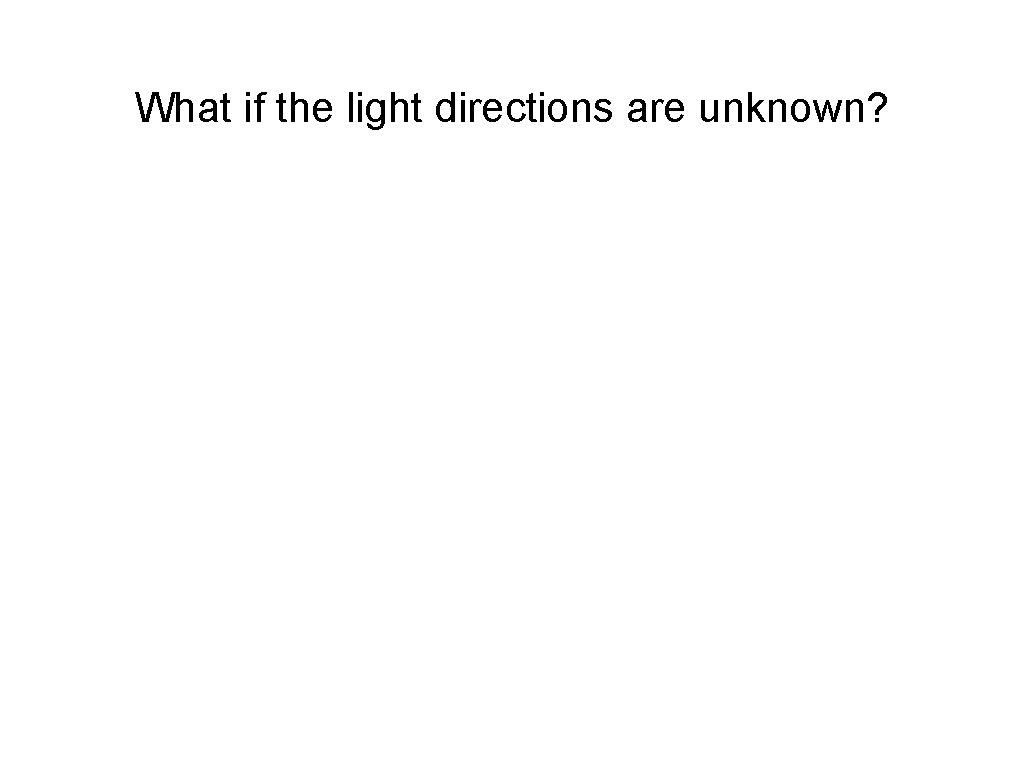What if the light directions are unknown?Uncalibrated photometric stereoWhat if the light directions are unknown? define “pseudo-normal” solve linear system for pseudo-normalWhat if the light directions are unknown? define “pseudo-normal” solve linear system for pseudo-normal at each image pixel M: number of pixelsWhat if the light directions are unknown? define “pseudo-normal” solve linear system for pseudo-normal at each image pixel How do we solve this system without knowing light matrix L?Factorizing the measurement matrix Lights Pseudonormals What are the dimensions?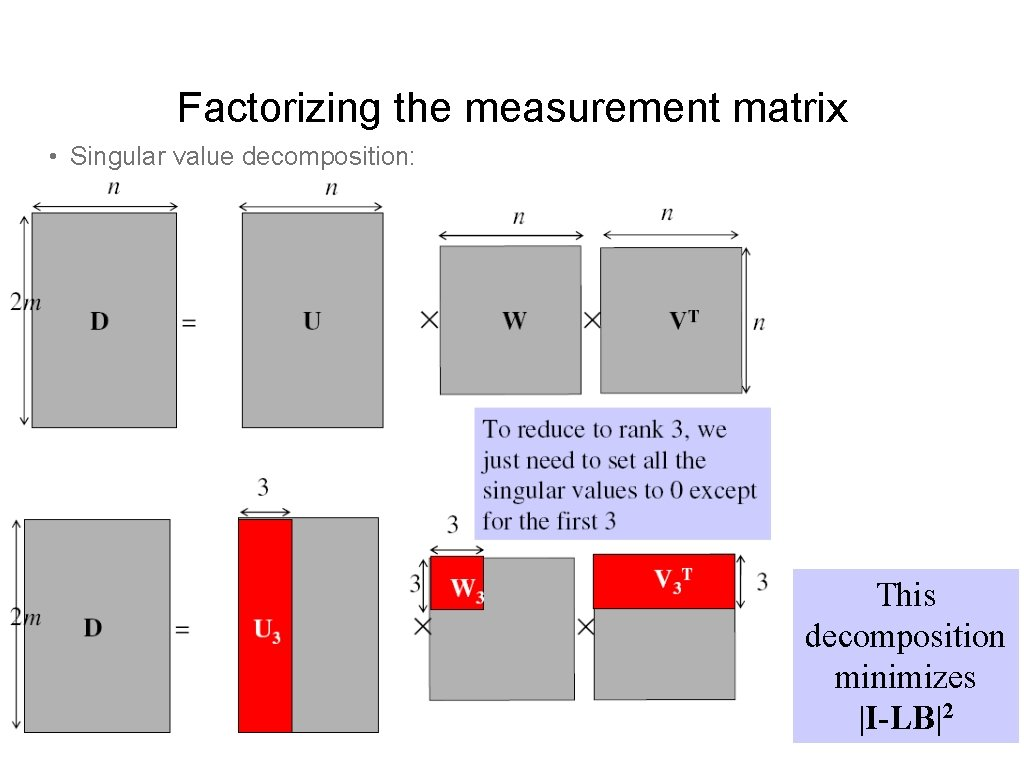Factorizing the measurement matrix • Singular value decomposition: This decomposition minimizes |I-LB|2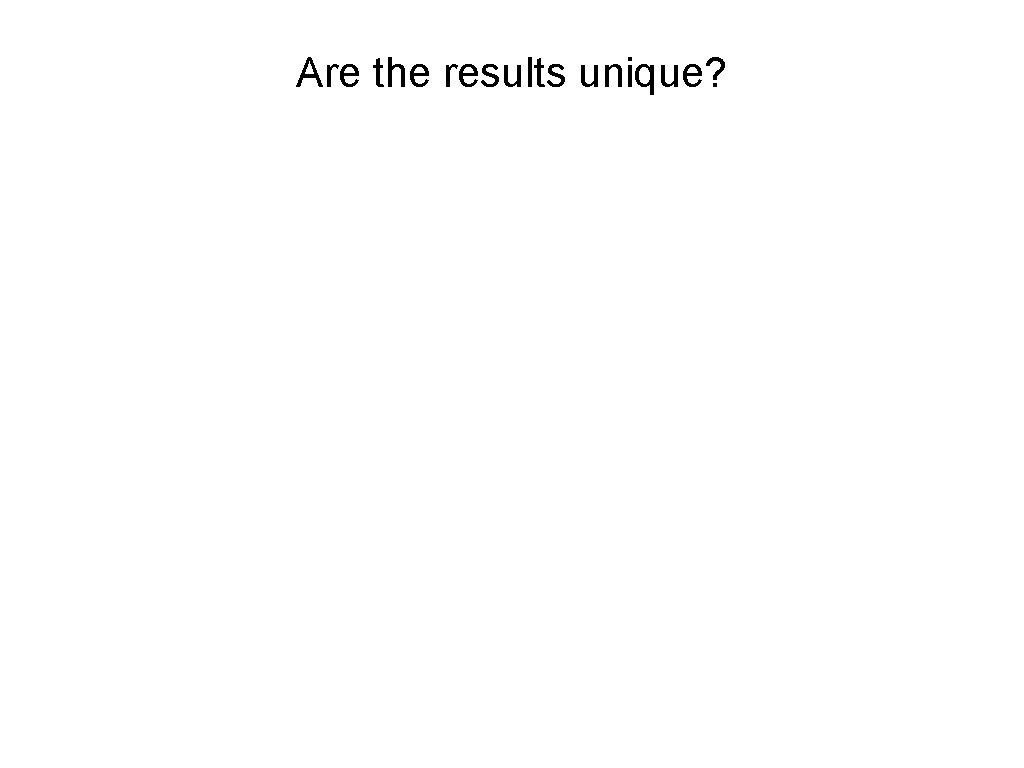Are the results unique?Are the results unique? We can insert any 3 x 3 matrix Q in the decomposition and get the same images: I = L B = (L Q-1) (Q B)Are the results unique? We can insert any 3 x 3 matrix Q in the decomposition and get the same images: I = L B = (L Q-1) (Q B) Can we use any assumptions to remove some of these 9 degrees of freedom?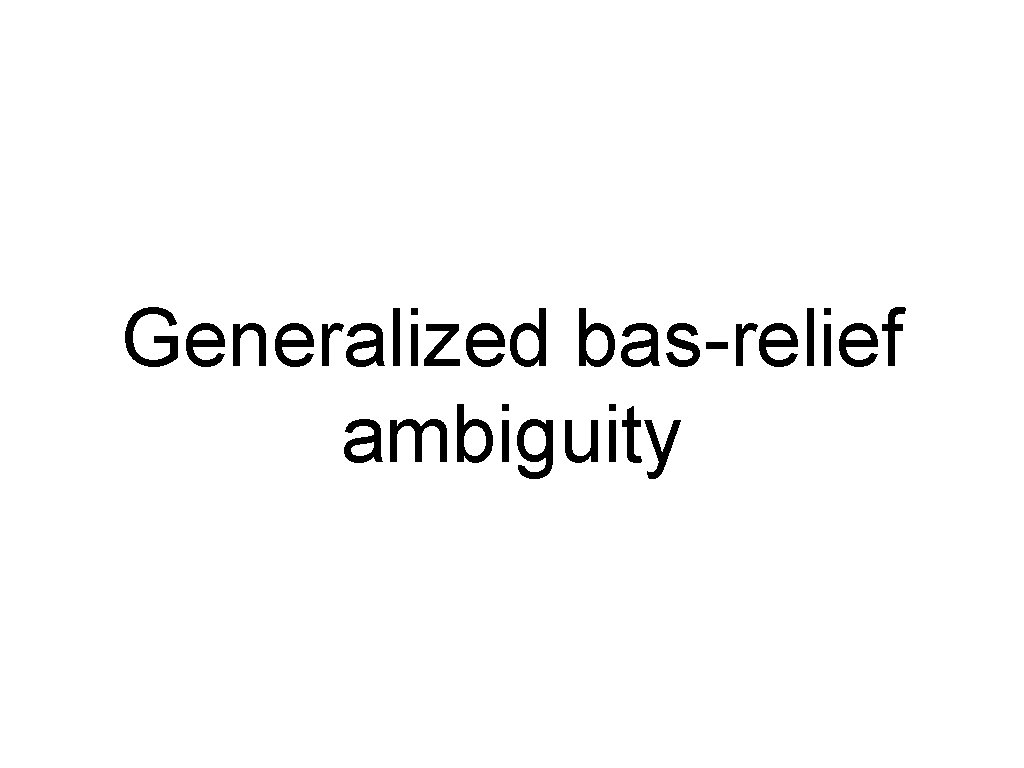Generalized bas-relief ambiguity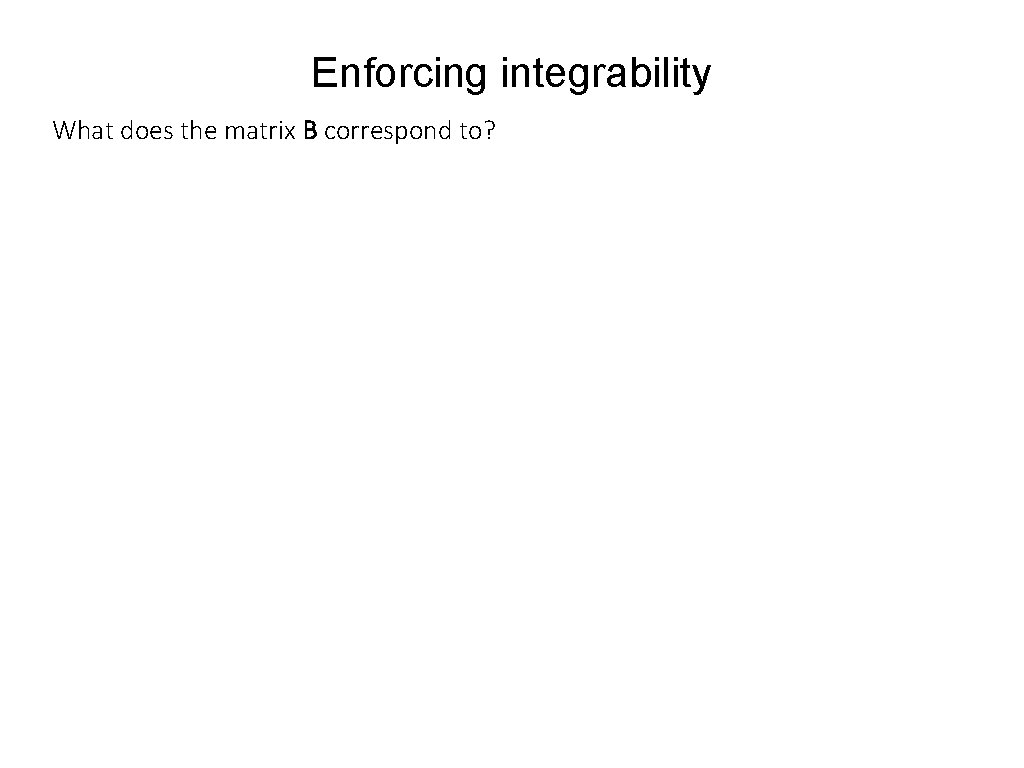Enforcing integrability What does the matrix B correspond to?Enforcing integrability What does the matrix B correspond to? • Surface representation as a depth image (also known as Monge surface): depth at each pixel • Unnormalized normal: pixel coordinates in image space • Actual normal: • Pseudo-normal: • Rearrange into 3 x. N matrix B.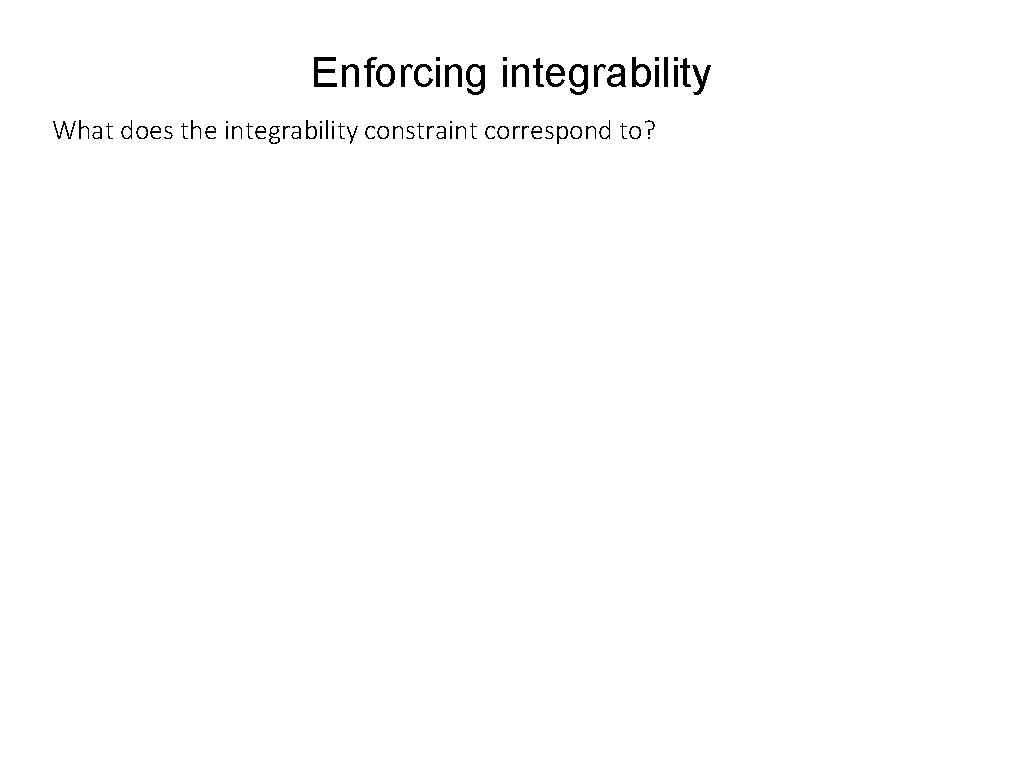Enforcing integrability What does the integrability constraint correspond to?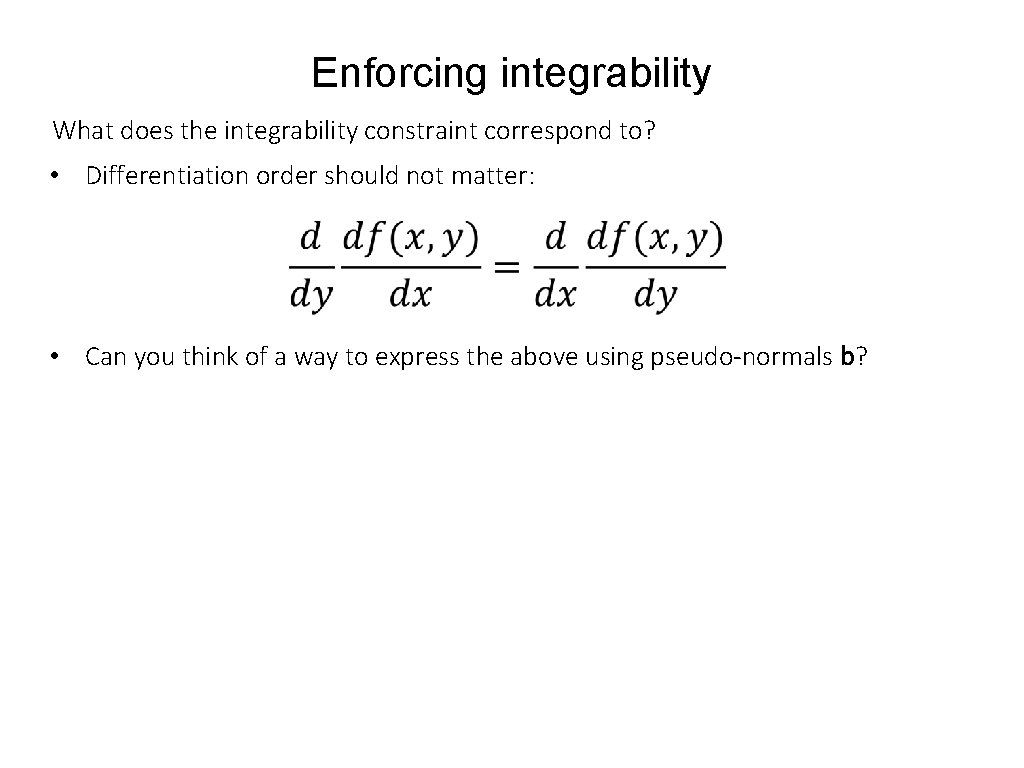Enforcing integrability What does the integrability constraint correspond to? • Differentiation order should not matter: • Can you think of a way to express the above using pseudo-normals b?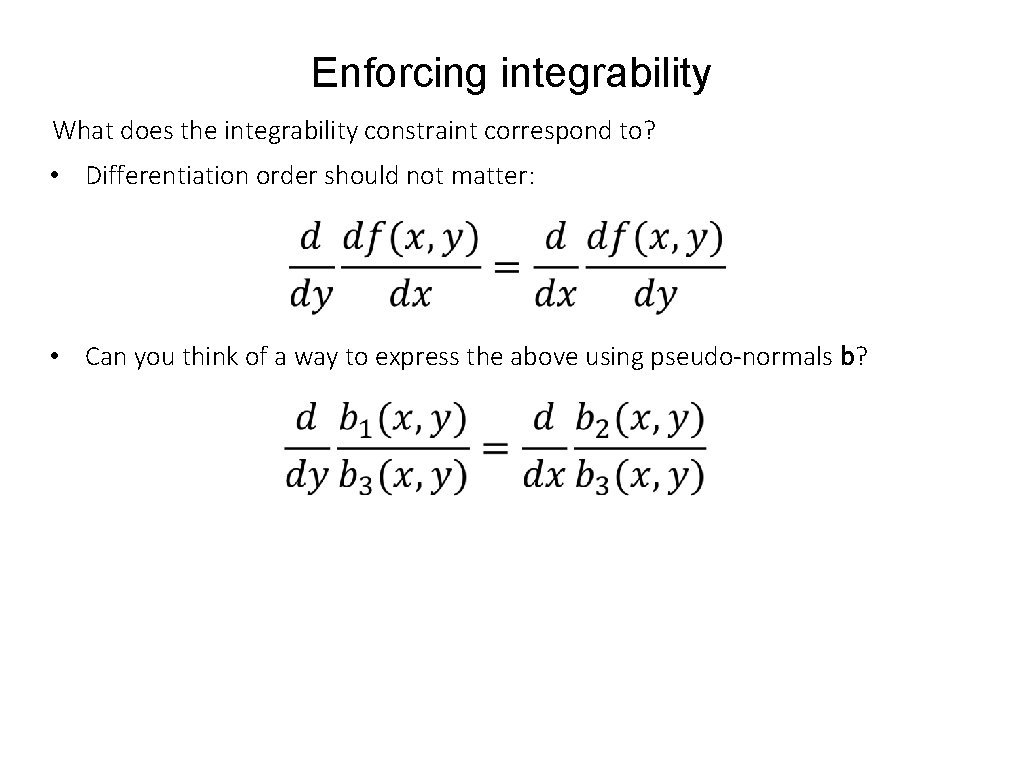Enforcing integrability What does the integrability constraint correspond to? • Differentiation order should not matter: • Can you think of a way to express the above using pseudo-normals b?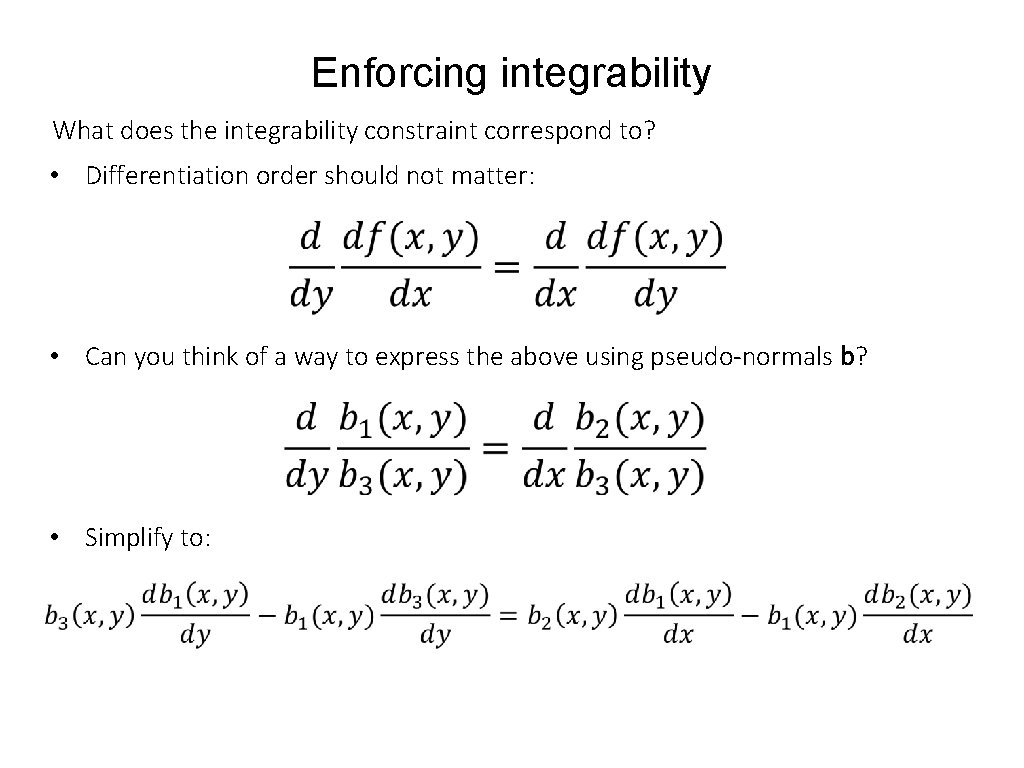Enforcing integrability What does the integrability constraint correspond to? • Differentiation order should not matter: • Can you think of a way to express the above using pseudo-normals b? • Simplify to:Enforcing integrability What does the integrability constraint correspond to? • Differentiation order should not matter: • Can you think of a way to express the above using pseudo-normals b? • Simplify to: • If Be is the pseudo-normal matrix we get from SVD, then find the 3 x 3 transform D such that B=D⋅Be is the closest to satisfying integrability in the least-squares sense.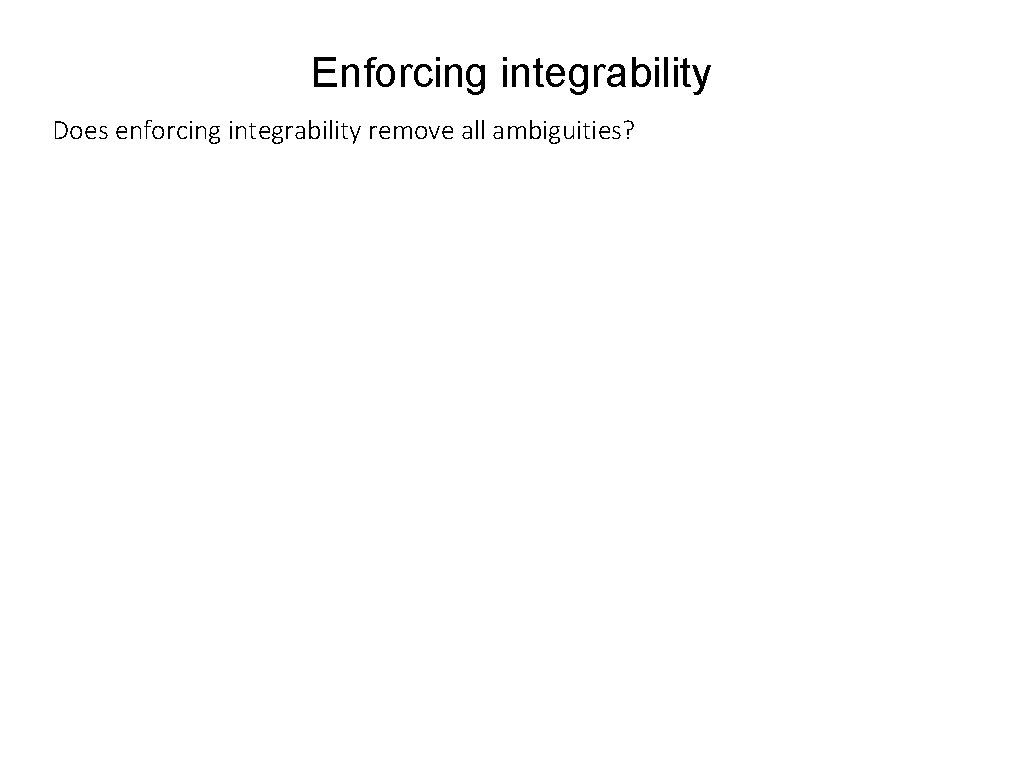Enforcing integrability Does enforcing integrability remove all ambiguities?Generalized Bas-relief ambiguity • Combined with transformed lights S’=G⋅S, the transformed pseudonormals produce the same images as the original pseudonormals. • This ambiguity cannot be removed using shadows. • This ambiguity can be removed using interreflections or additional assumptions. This ambiguity is known as the generalized bas-relief ambiguity.Generalized Bas-relief ambiguity Otherwise, includes shearing.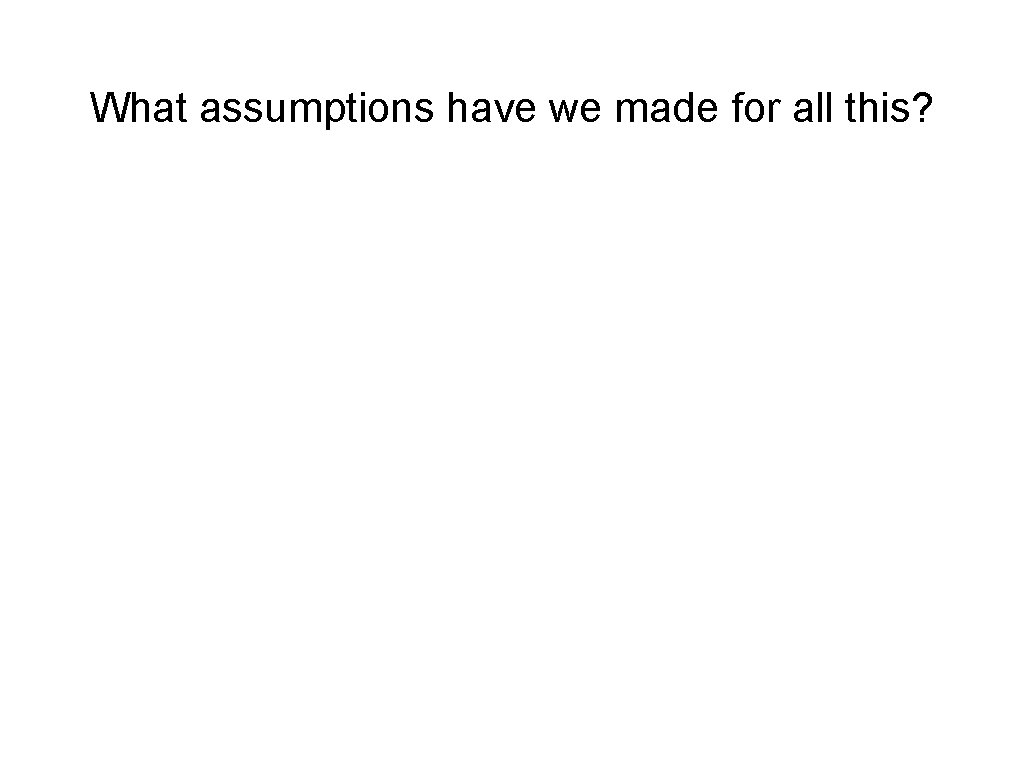What assumptions have we made for all this?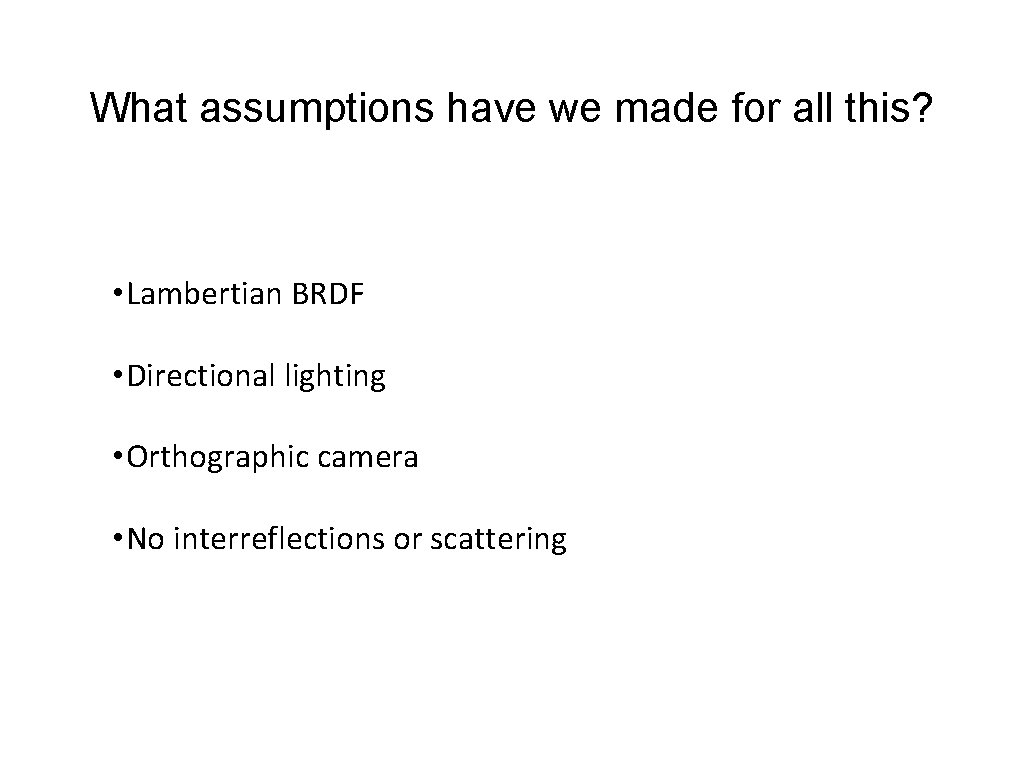What assumptions have we made for all this? • Lambertian BRDF • Directional lighting • Orthographic camera • No interreflections or scattering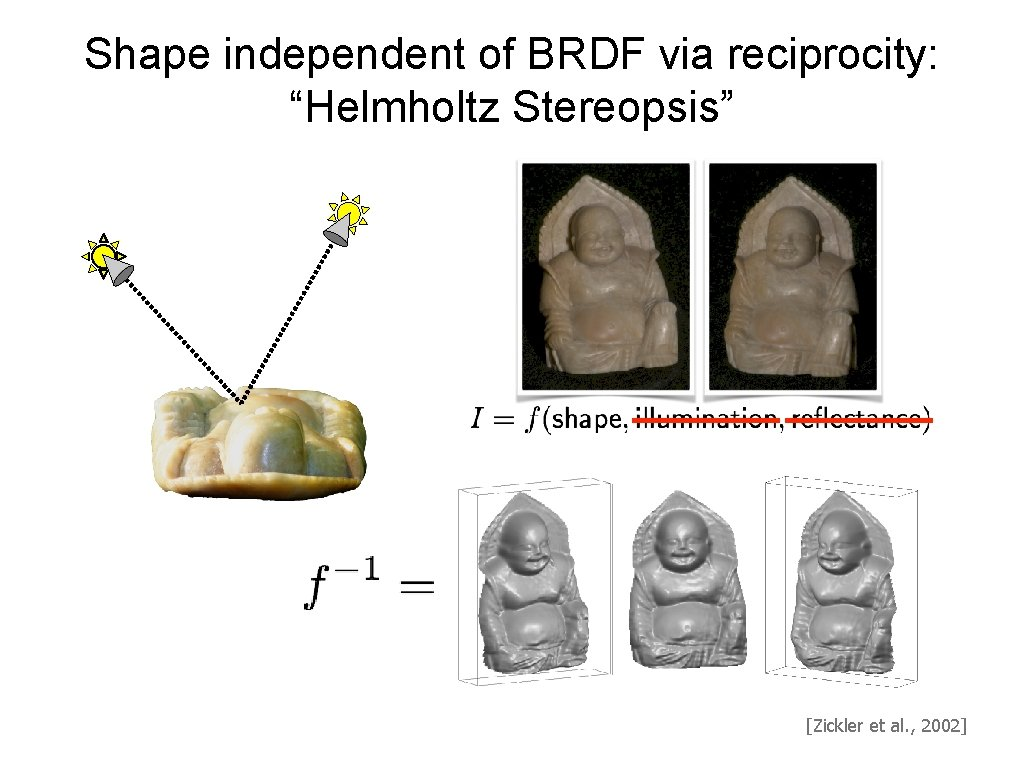Shape independent of BRDF via reciprocity: “Helmholtz Stereopsis” [Zickler et al. , 2002]Can we reconstruct shape from one image?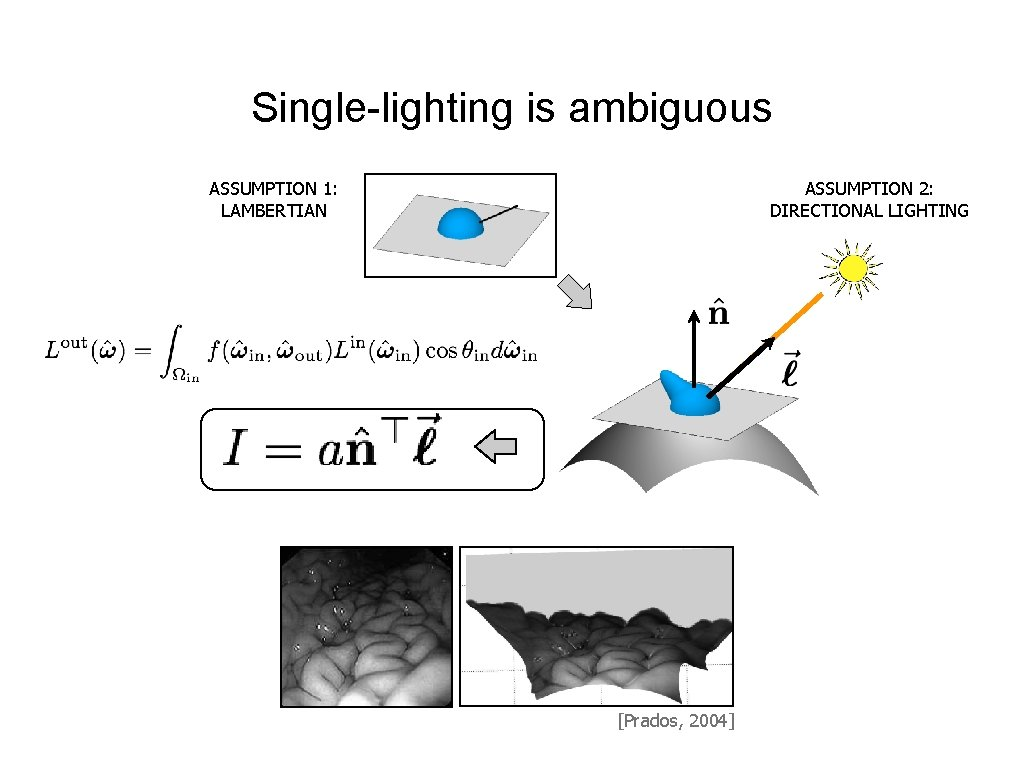Single-lighting is ambiguous ASSUMPTION 1: LAMBERTIAN ASSUMPTION 2: DIRECTIONAL LIGHTING [Prados, 2004]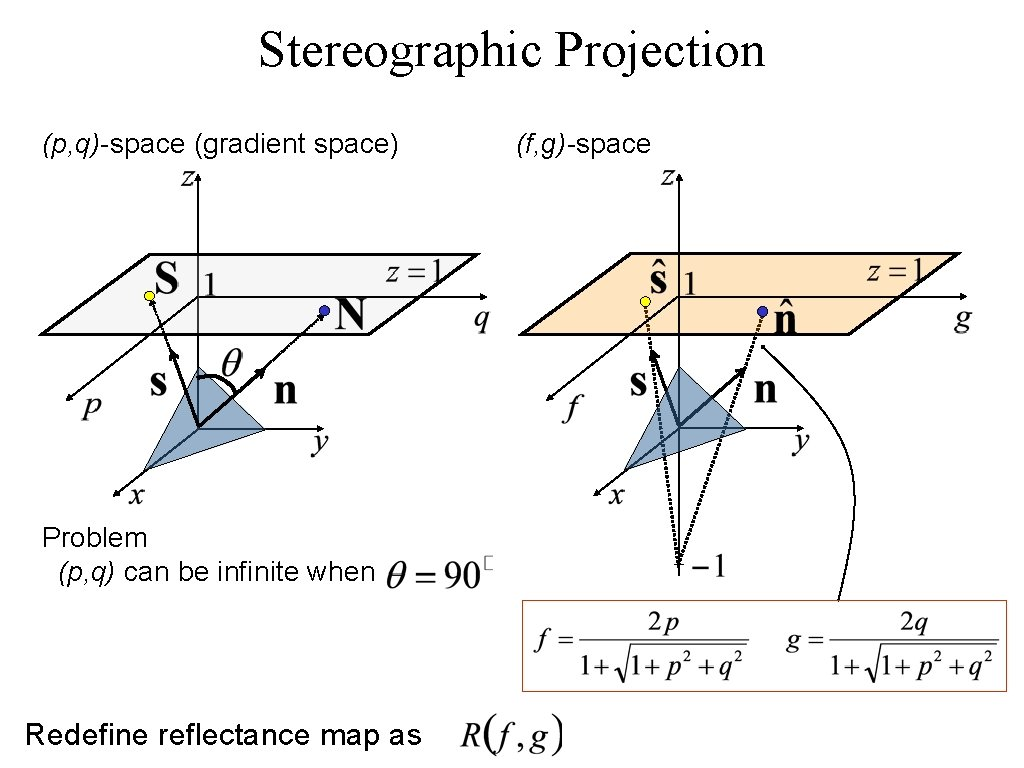Stereographic Projection (p, q)-space (gradient space) Problem (p, q) can be infinite when Redefine reflectance map as (f, g)-space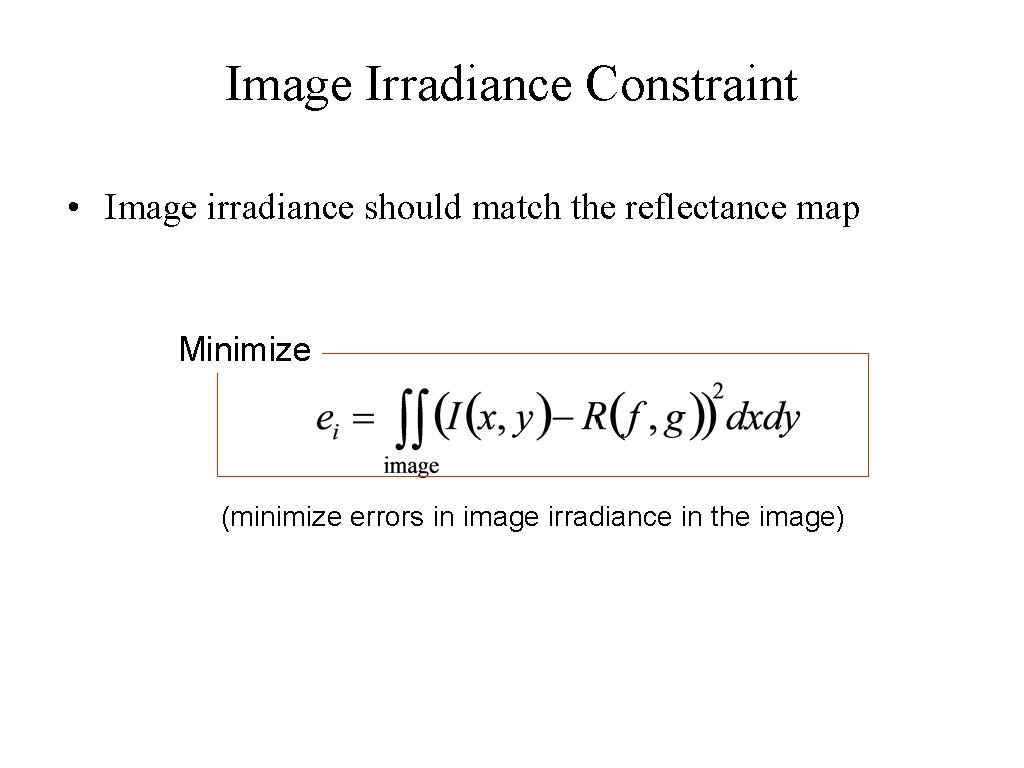Image Irradiance Constraint • Image irradiance should match the reflectance map Minimize (minimize errors in image irradiance in the image)Smoothness Constraint • Used to constrain shape-from-shading • Relates orientations (f, g) of neighboring surface points Minimize : surface orientation under stereographic projection (penalize rapid changes in surface orientation f and g over the image)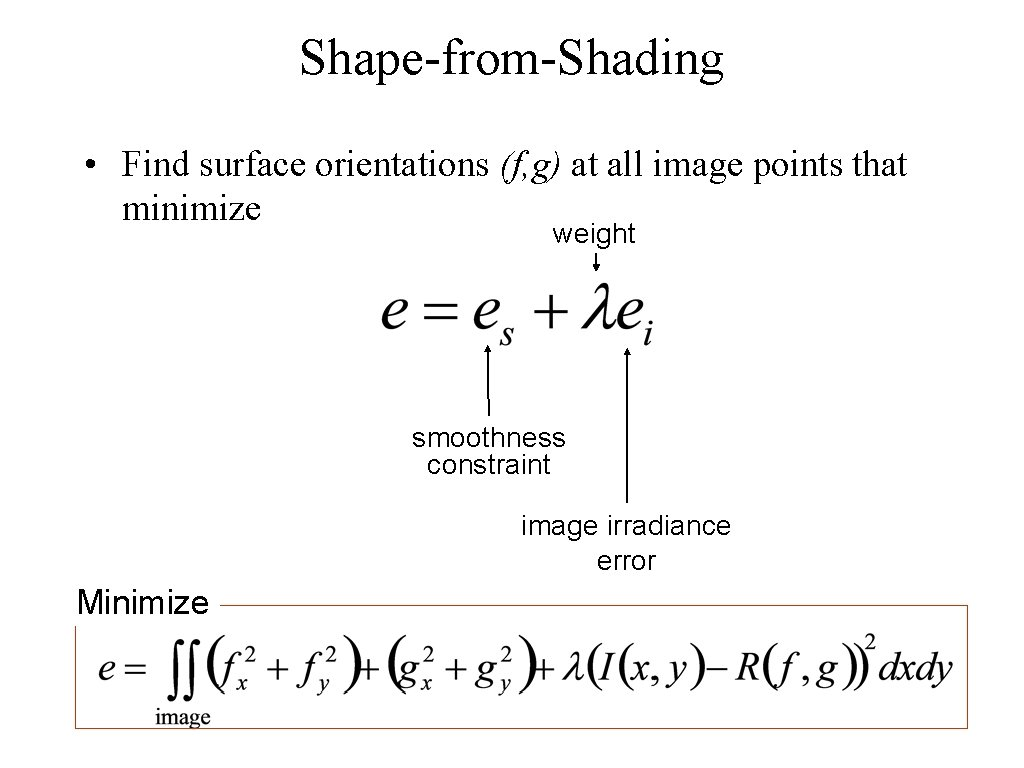Shape-from-Shading • Find surface orientations (f, g) at all image points that minimize weight smoothness constraint image irradiance error Minimize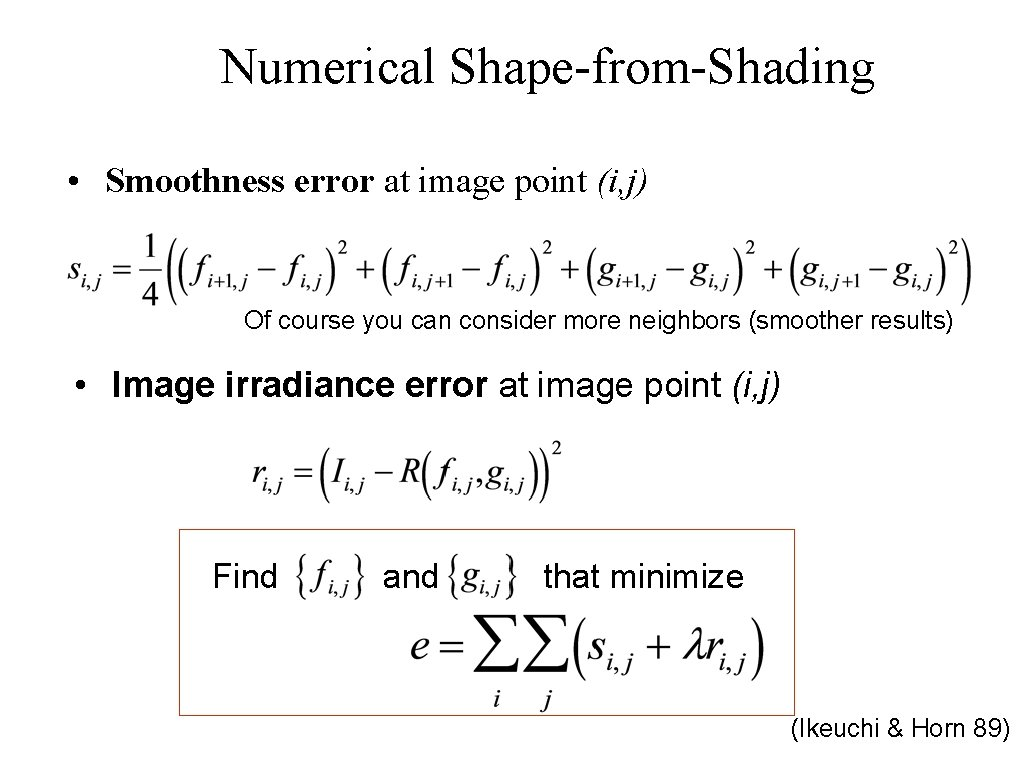Numerical Shape-from-Shading • Smoothness error at image point (i, j) Of course you can consider more neighbors (smoother results) • Image irradiance error at image point (i, j) Find and that minimize (Ikeuchi & Horn 89)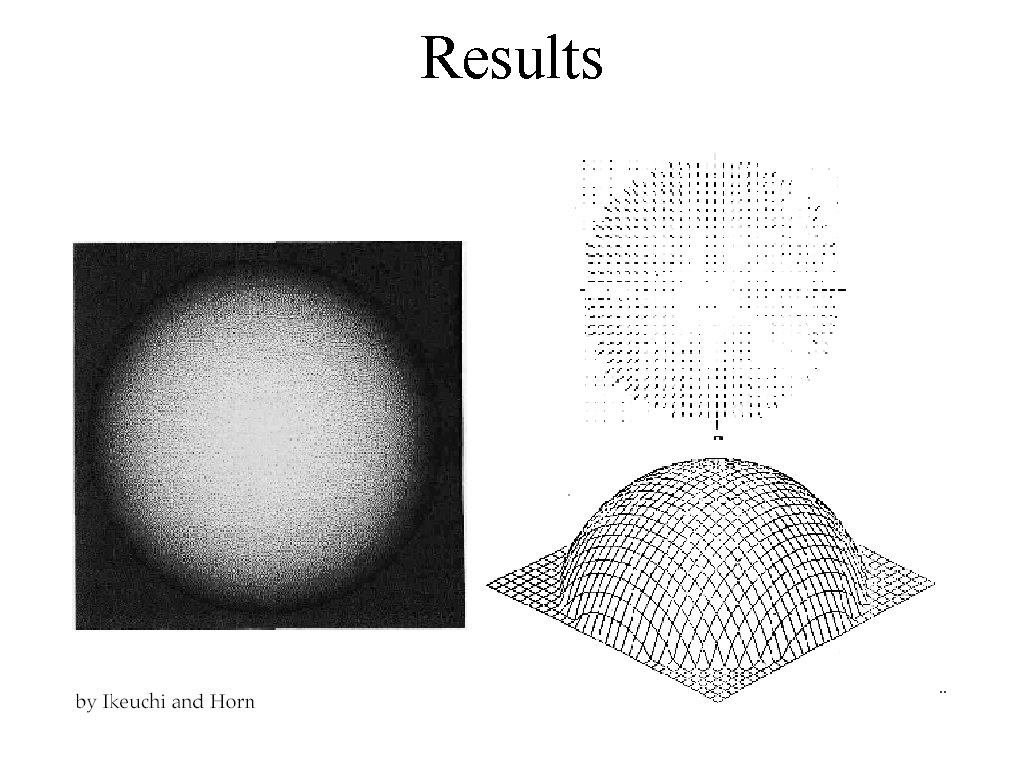Results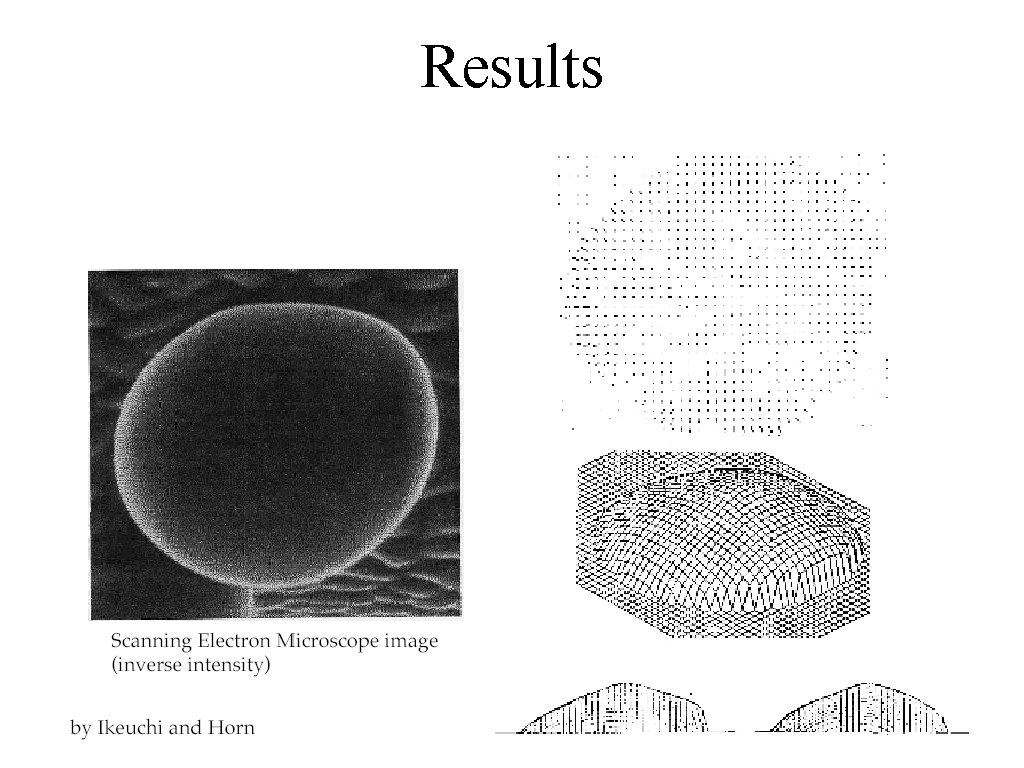ResultsMore modern results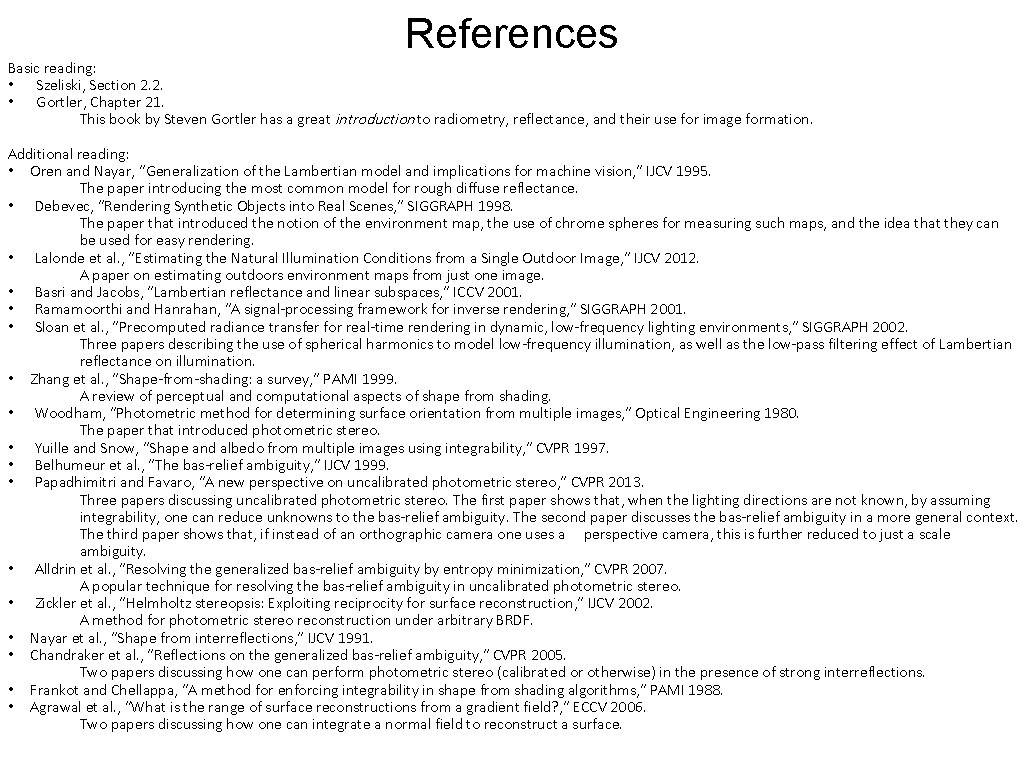References Basic reading: • Szeliski, Section 2. 2. • Gortler, Chapter 21. This book by Steven Gortler has a great introduction to radiometry, reflectance, and their use for image formation. Additional reading: • Oren and Nayar, “Generalization of the Lambertian model and implications for machine vision, ” IJCV 1995. The paper introducing the most common model for rough diffuse reflectance. • Debevec, “Rendering Synthetic Objects into Real Scenes, ” SIGGRAPH 1998. The paper that introduced the notion of the environment map, the use of chrome spheres for measuring such maps, and the idea that they can be used for easy rendering. • Lalonde et al. , “Estimating the Natural Illumination Conditions from a Single Outdoor Image, ” IJCV 2012. A paper on estimating outdoors environment maps from just one image. • Basri and Jacobs, “Lambertian reflectance and linear subspaces, ” ICCV 2001. • Ramamoorthi and Hanrahan, “A signal-processing framework for inverse rendering, ” SIGGRAPH 2001. • Sloan et al. , “Precomputed radiance transfer for real-time rendering in dynamic, low-frequency lighting environments, ” SIGGRAPH 2002. Three papers describing the use of spherical harmonics to model low-frequency illumination, as well as the low-pass filtering effect of Lambertian reflectance on illumination. • Zhang et al. , “Shape-from-shading: a survey, ” PAMI 1999. A review of perceptual and computational aspects of shape from shading. • Woodham, “Photometric method for determining surface orientation from multiple images, ” Optical Engineering 1980. The paper that introduced photometric stereo. • Yuille and Snow, “Shape and albedo from multiple images using integrability, ” CVPR 1997. • Belhumeur et al. , “The bas-relief ambiguity, ” IJCV 1999. • Papadhimitri and Favaro, “A new perspective on uncalibrated photometric stereo, ” CVPR 2013. Three papers discussing uncalibrated photometric stereo. The first paper shows that, when the lighting directions are not known, by assuming integrability, one can reduce unknowns to the bas-relief ambiguity. The second paper discusses the bas-relief ambiguity in a more general context. The third paper shows that, if instead of an orthographic camera one uses a perspective camera, this is further reduced to just a scale ambiguity. • Alldrin et al. , “Resolving the generalized bas-relief ambiguity by entropy minimization, ” CVPR 2007. A popular technique for resolving the bas-relief ambiguity in uncalibrated photometric stereo. • Zickler et al. , “Helmholtz stereopsis: Exploiting reciprocity for surface reconstruction, ” IJCV 2002. A method for photometric stereo reconstruction under arbitrary BRDF. • Nayar et al. , “Shape from interreflections, ” IJCV 1991. • Chandraker et al. , “Reflections on the generalized bas-relief ambiguity, ” CVPR 2005. Two papers discussing how one can perform photometric stereo (calibrated or otherwise) in the presence of strong interreflections. • Frankot and Chellappa, “A method for enforcing integrability in shape from shading algorithms, ” PAMI 1988. • Agrawal et al. , “What is the range of surface reconstructions from a gradient field? , ” ECCV 2006. Two papers discussing how one can integrate a normal field to reconstruct a surface.